﻿ Free 3rd Grade Math Worksheets PDF

Page No. 1006

Math / Math Worksheets / 3rd Grade Math Worksheets

# Free 3rd Grade Math Worksheets PDF

Download free printable math worksheets for 3rd grade in PDF. Our math worksheets for Grade 3 can be used in the classroom or for home practice. These math 3rd Grade worksheets contain also a link to the online game which helps students to improve their 3rd Grade math skills.

You can also try our Online Math Games for Grade 3 here →

### Add and subtract to 100

Mixed addition and subtraction puzzles up to 100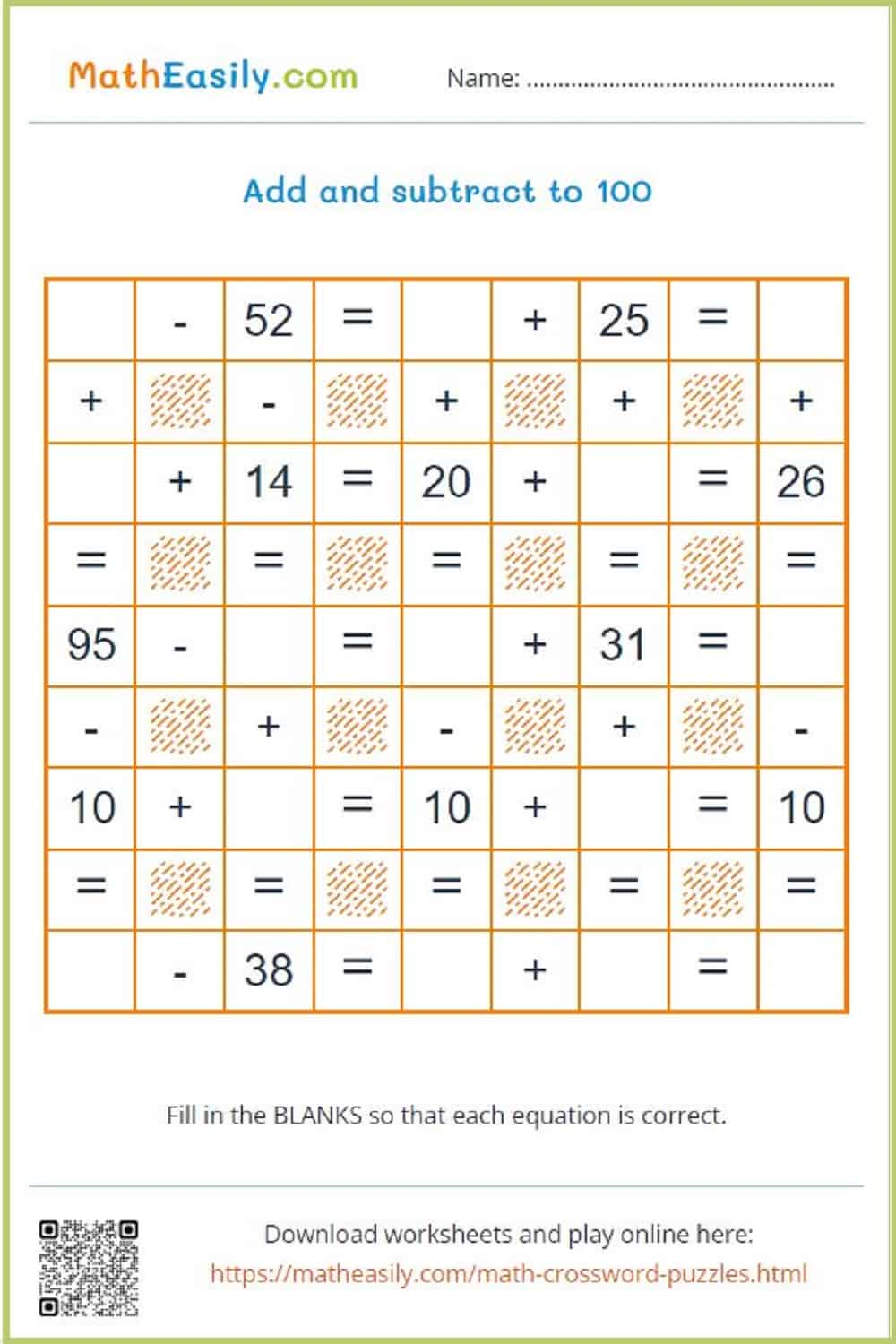Generate a new PDF

### Emoji maths puzzlesGenerate a new PDF

### Multiplication crossword

Generate multiplication crossword puzzles in PDF.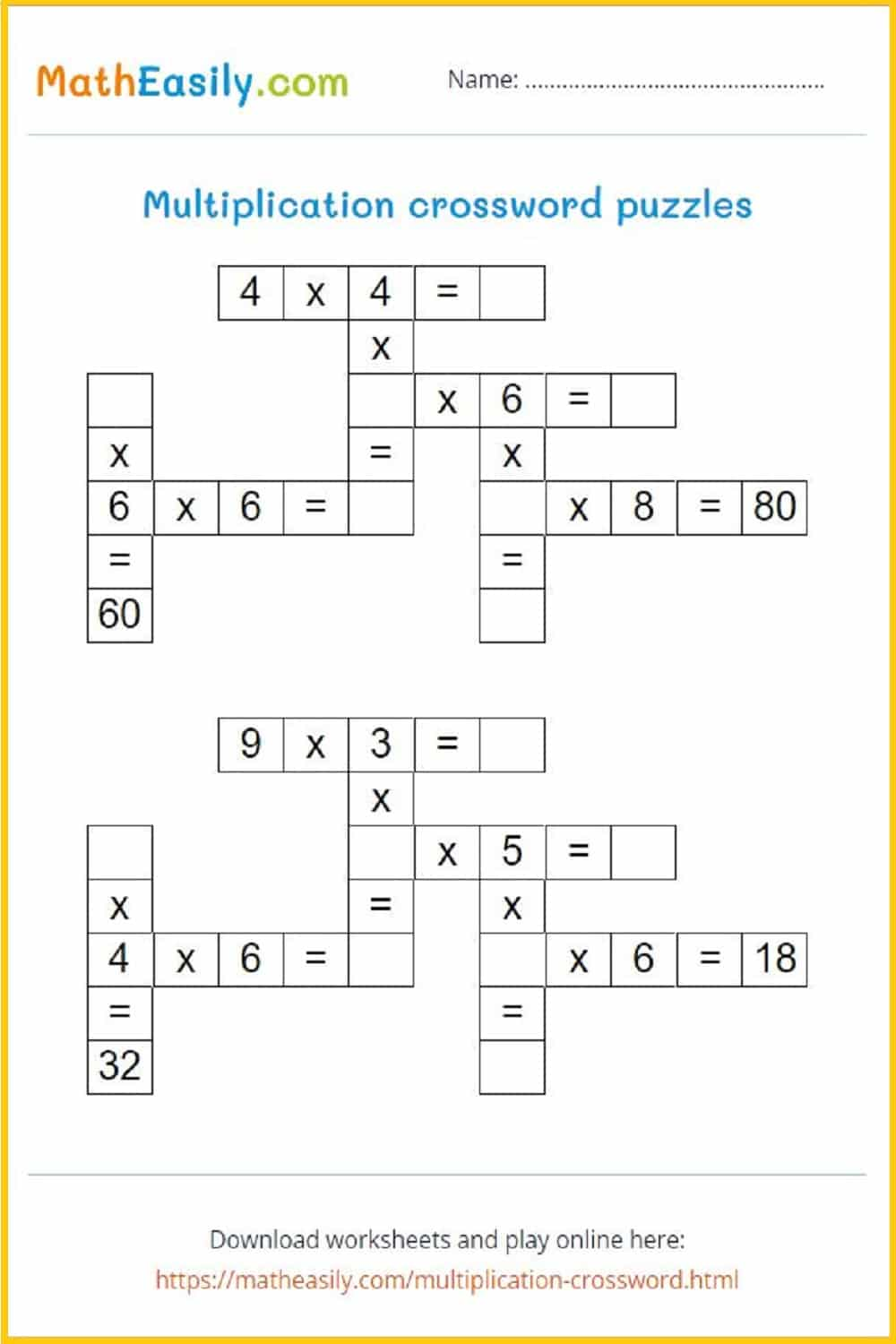Generate a new PDF

### Magic squares puzzles

Magic squares puzzles: math worksheets for 3rd grade.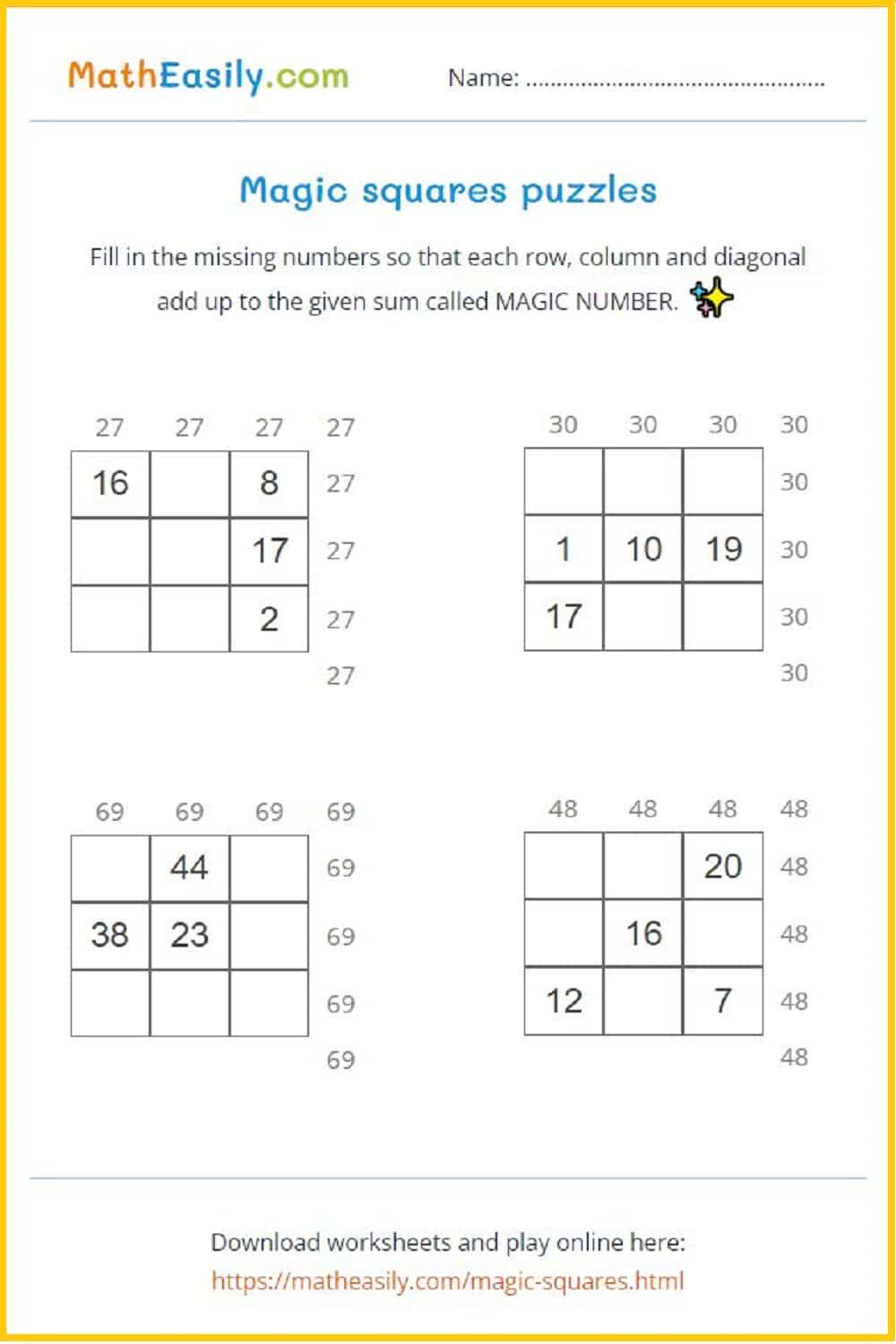Generate a new PDF

## Free Printable 3rd Grade Math Worksheets PDF

Most of these 3rd grade math worksheets are generated automatically, so every time you get a new worksheet. So, if there is written ´Generate a new PDF´ under the worksheet, you can download as many different free math worksheets for 3rd grade as you want.

### Class timetable

School timetable template in PDF and EXCEL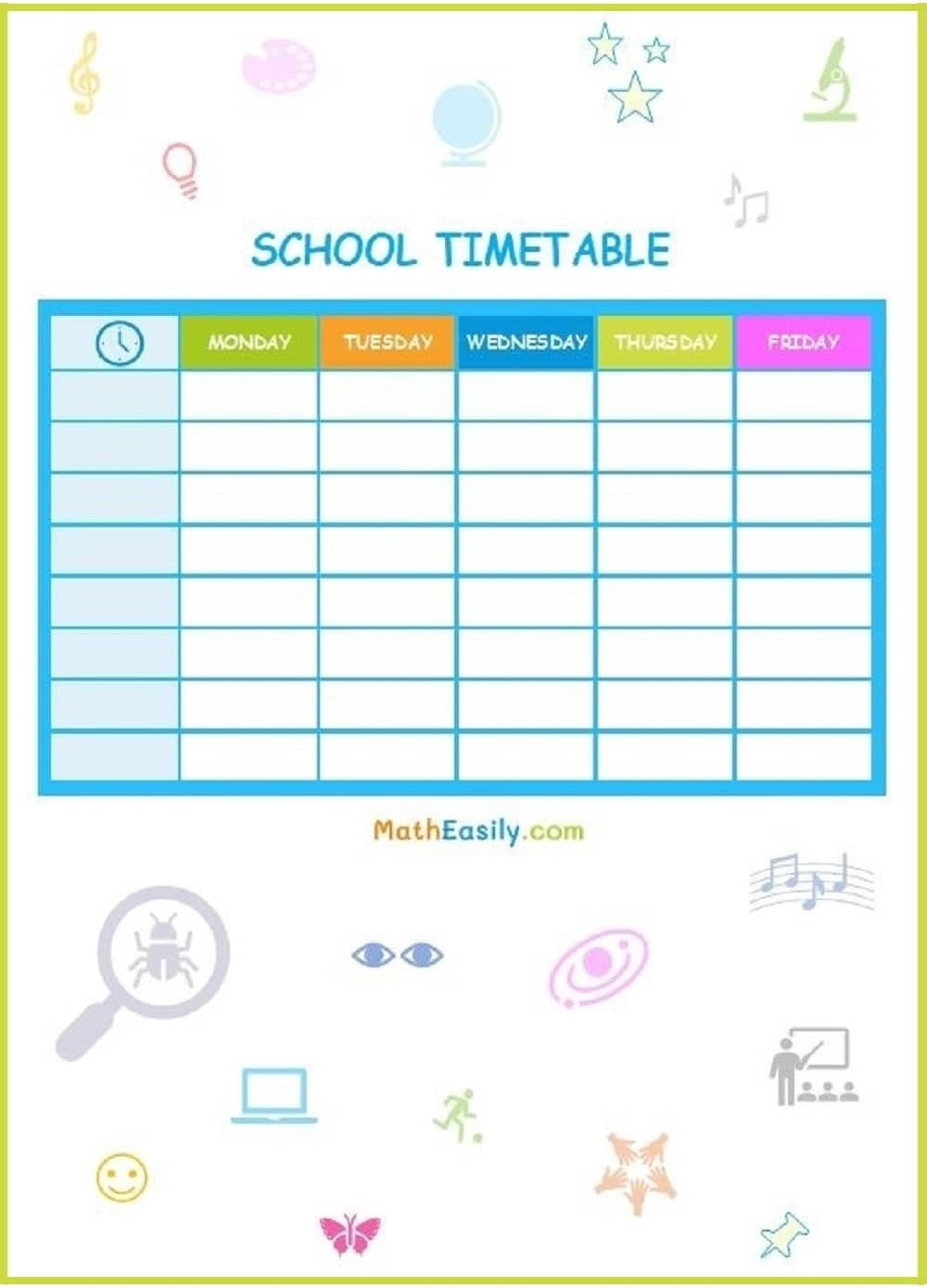2 digit addition worksheets WITHOUT regroupingGenerate a new PDF

2 digit addition worksheets WITH regrouping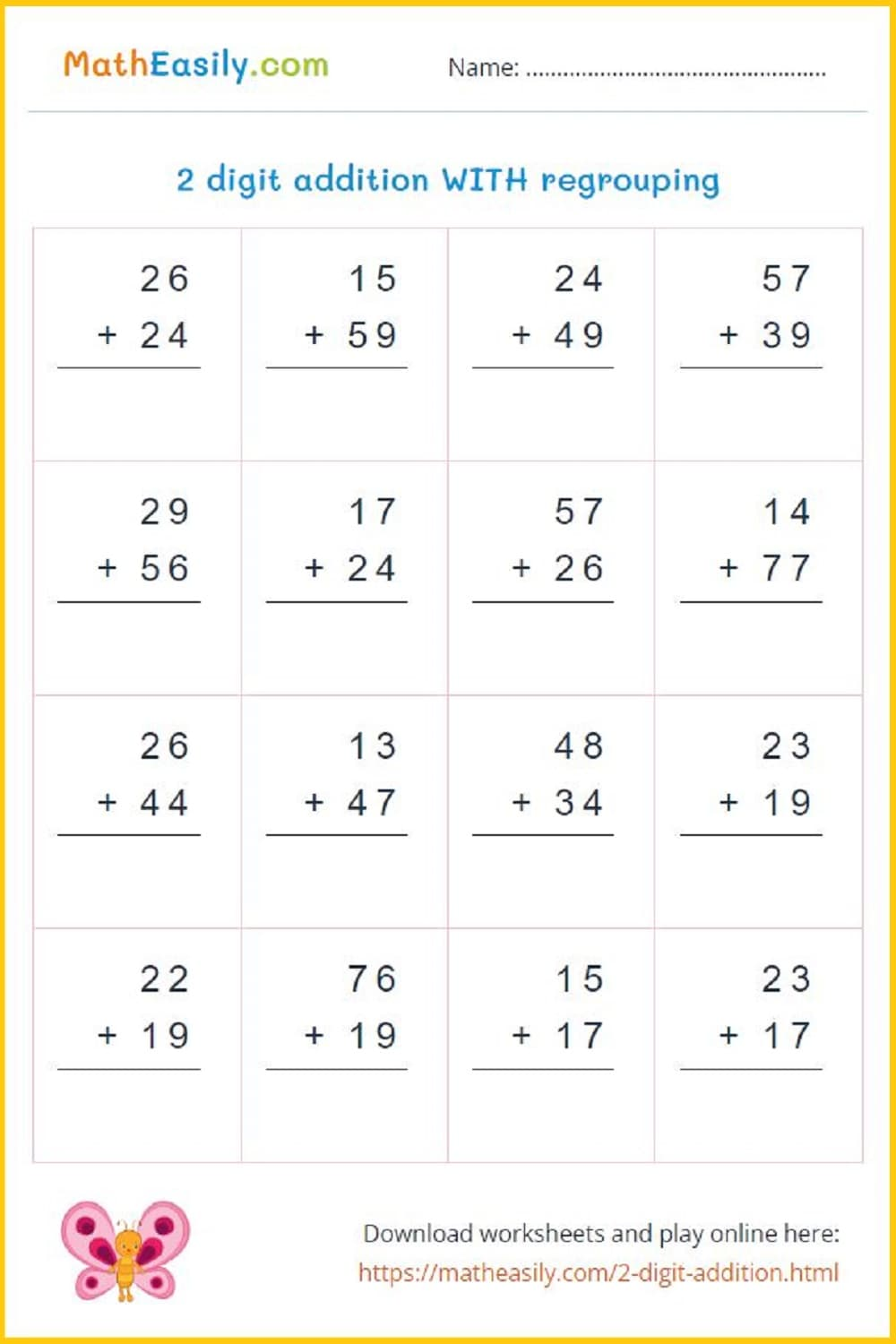Generate a new PDF

### Identifying fractions

What is the fraction of the shaded part?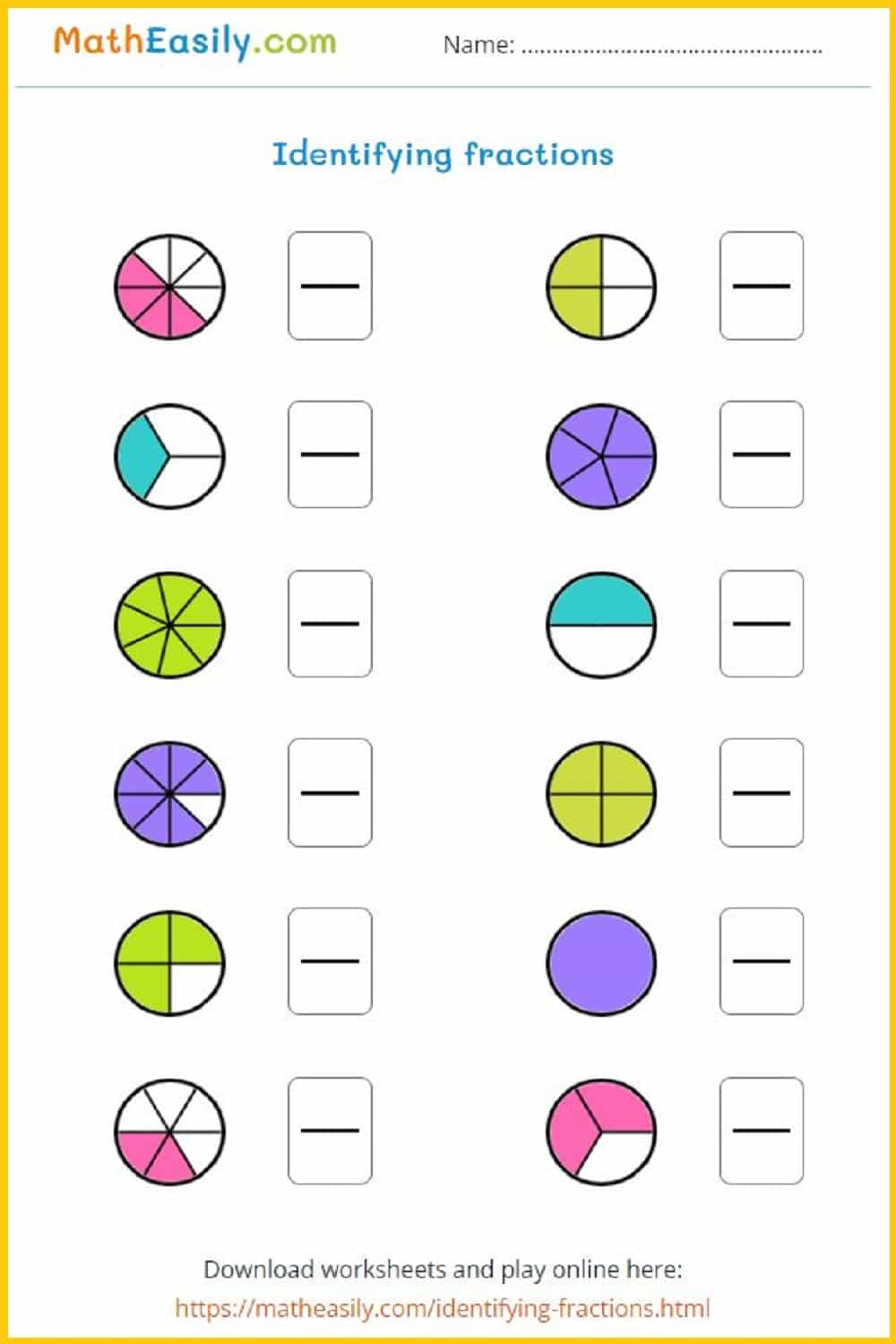Generate a new PDF

### Fraction identification

Color each circle to show the equivalent fraction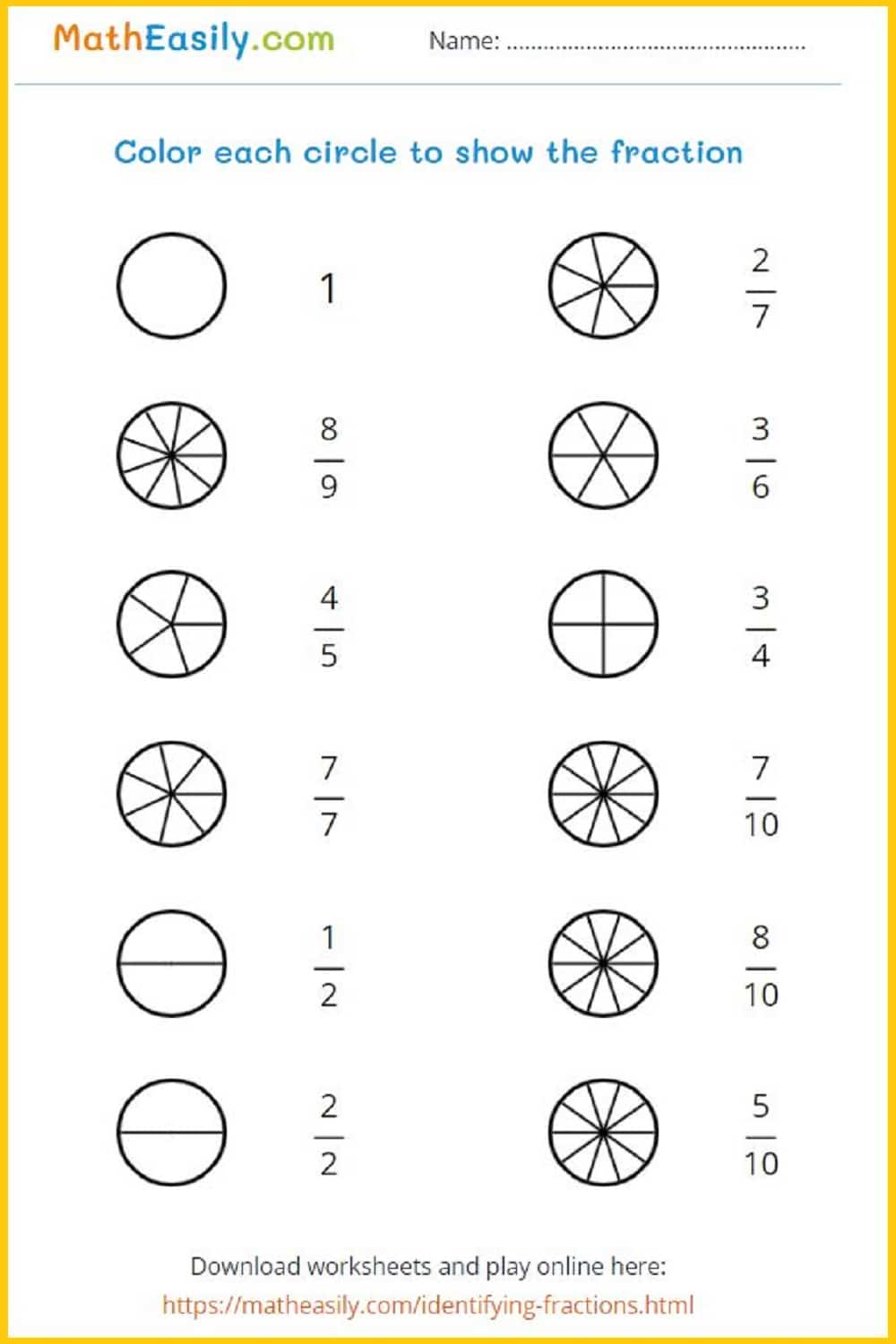Generate a new PDF

### Number bonds to 100

Generate number bonds to 100: worksheets in PDF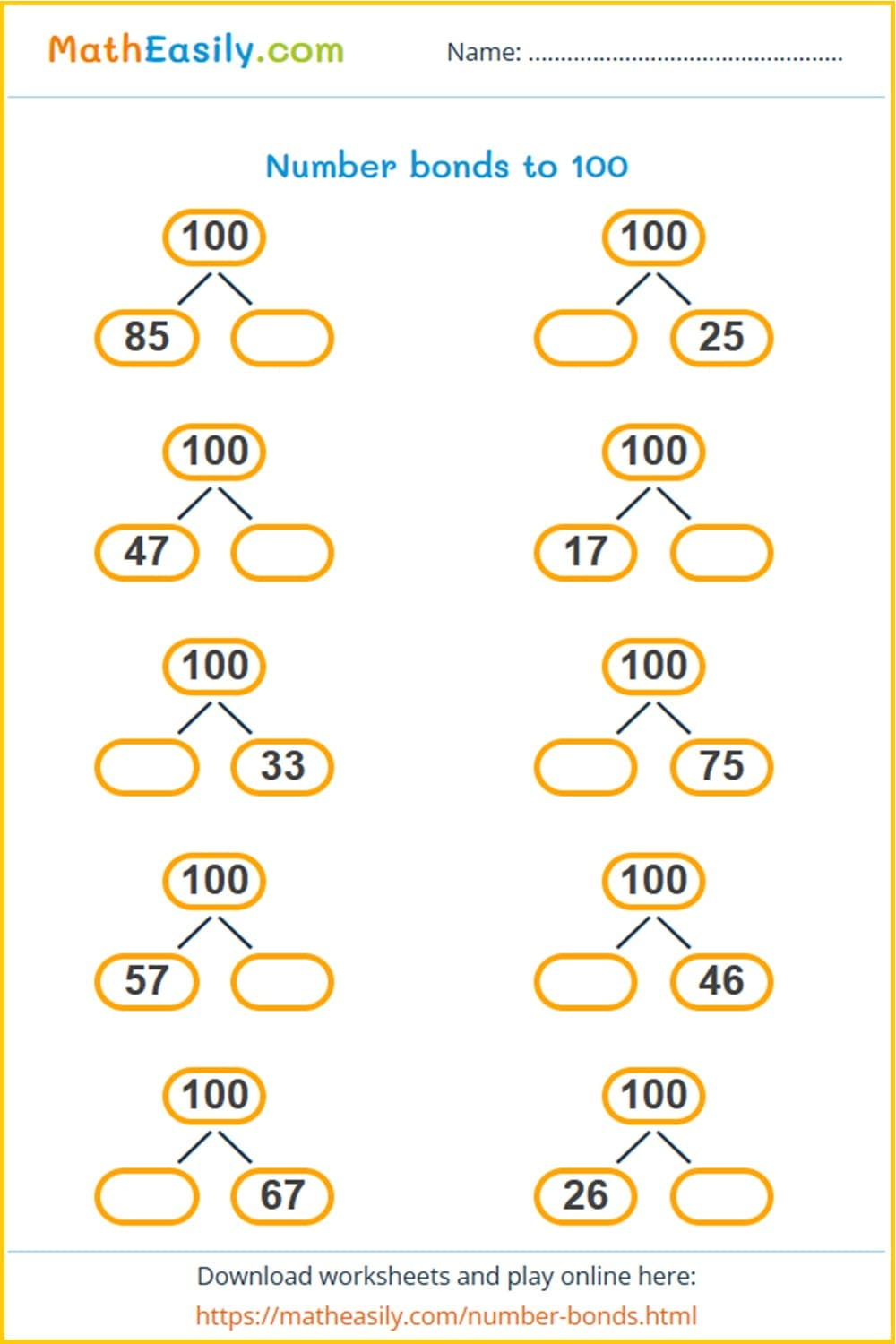Generate a new PDF

### Christmas tree

Fill in the christmas tree pyramid puzzles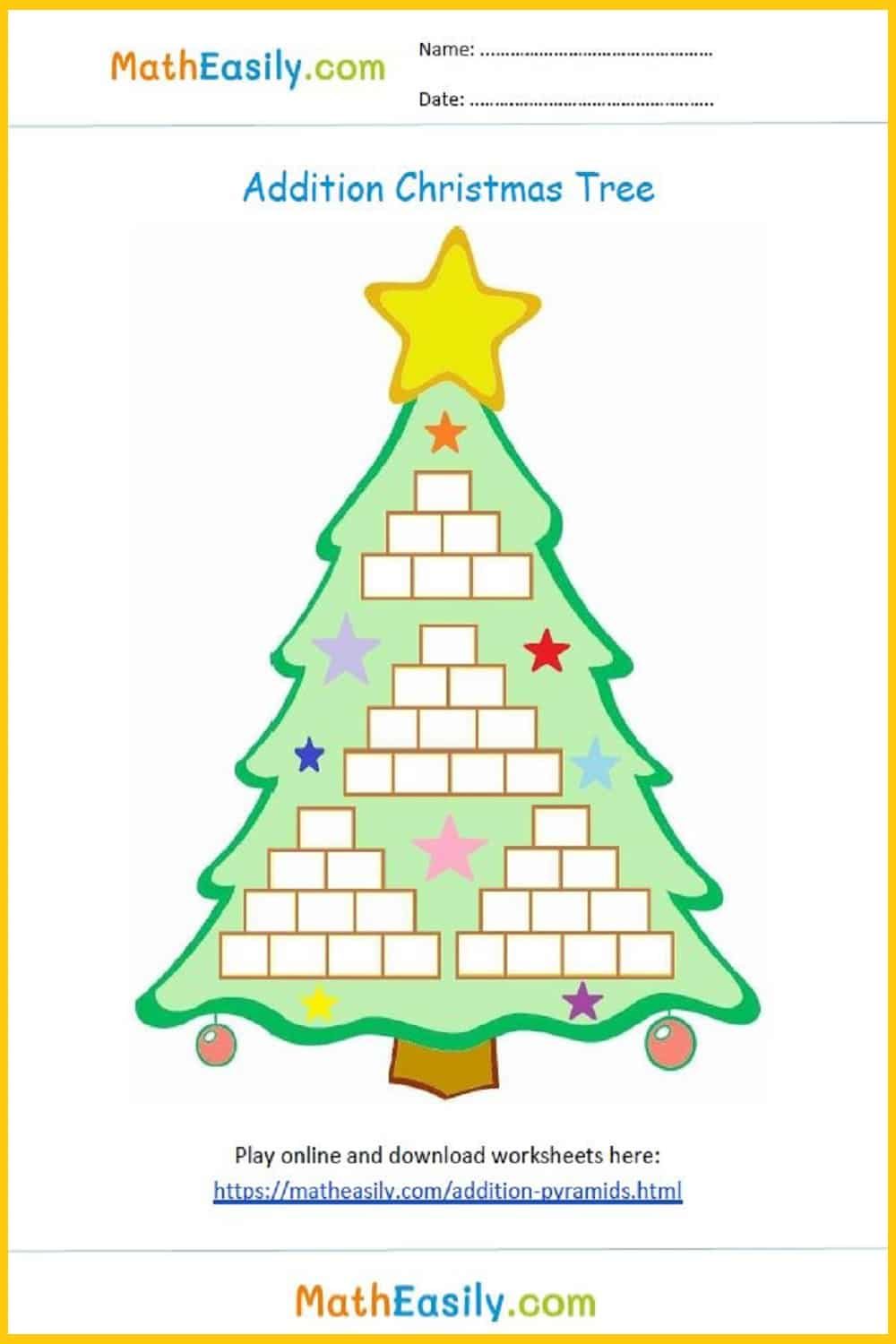### Subtraction pyramids

Fill in subtraction pyramid puzzles up to 100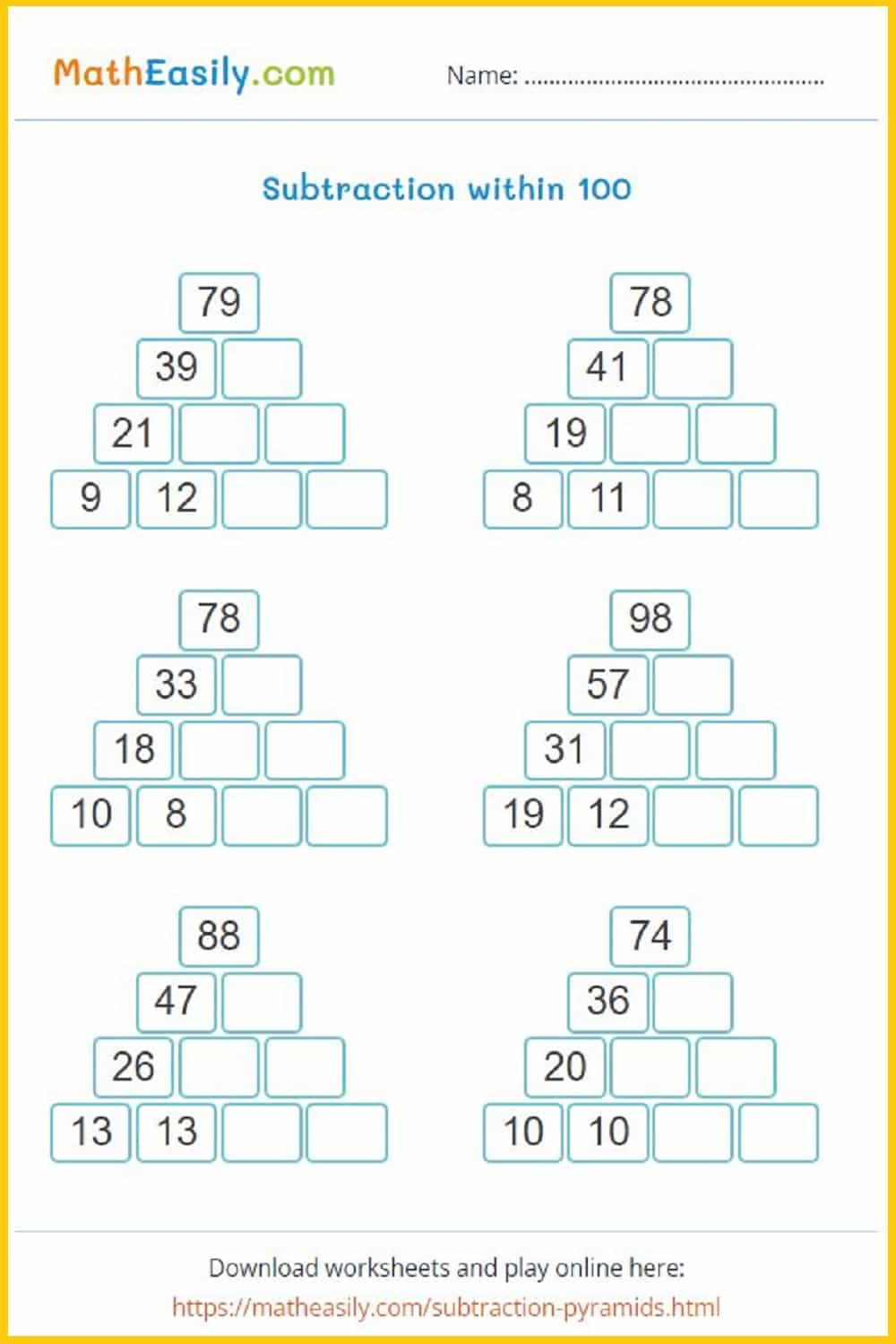Generate a new PDF

### 100 Math Puzzles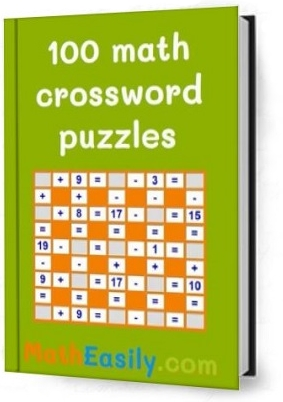Look inside here

### Add and subtract to 100

Mixed addition and subtraction puzzles up to 100Generate a new PDF

### 2 digit subtraction

2 digit subtraction without regrouping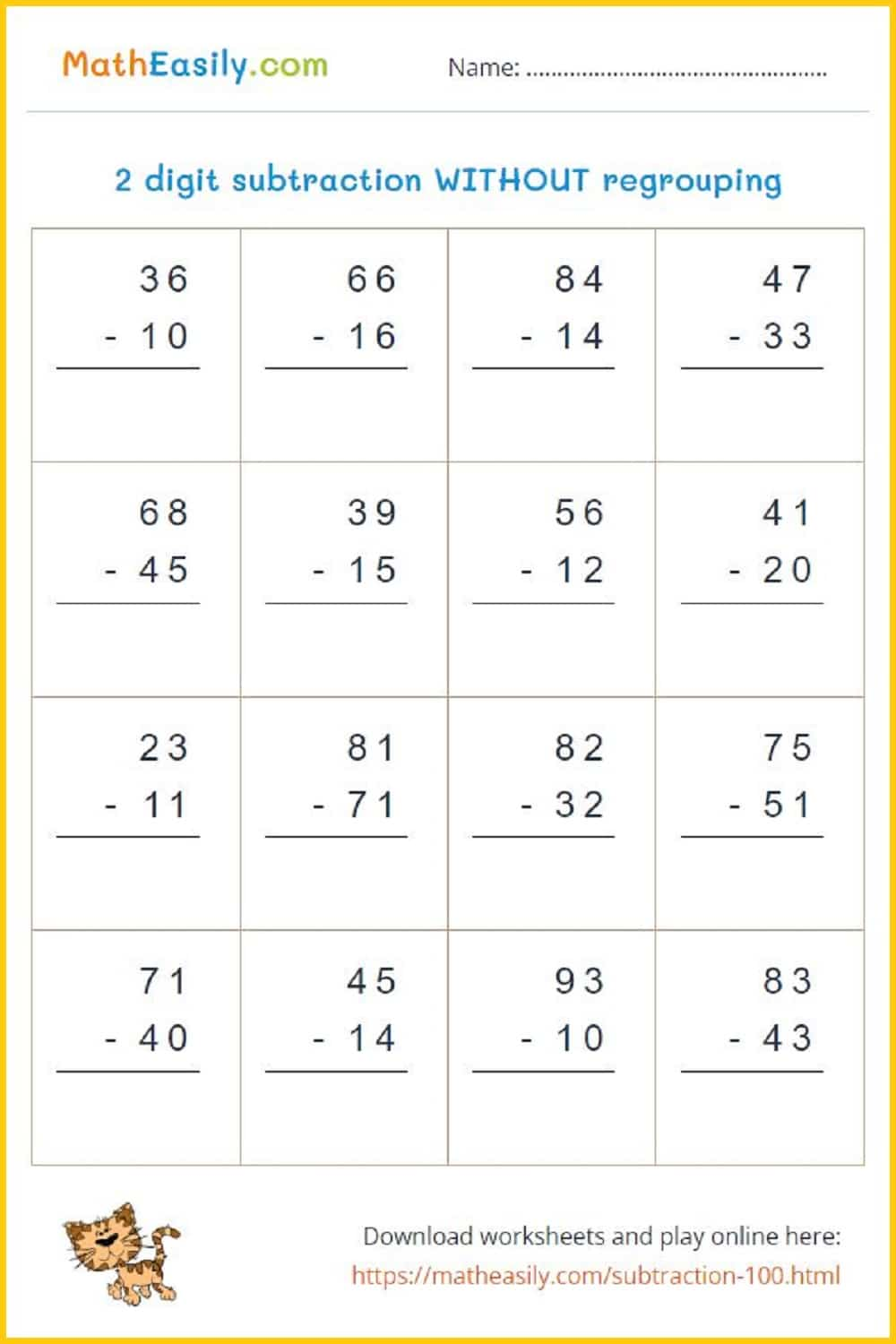Generate a new PDF

### 2 digit subtraction

2 digit subtraction worksheets: with regrouping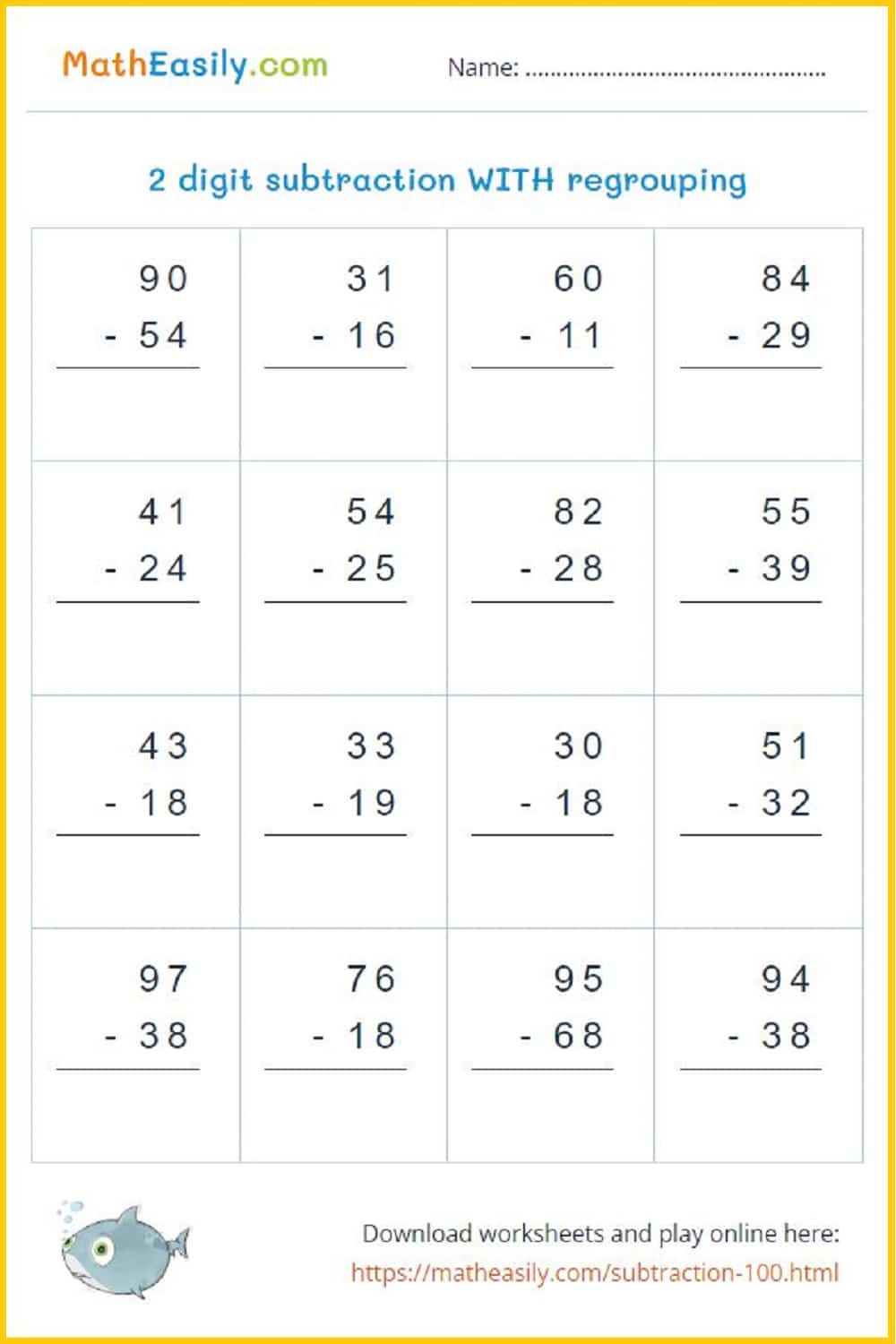Generate a new PDF

3 digit addition worksheets WITHOUT regroupingGenerate a new PDF

3 digit addition worksheets WITH regrouping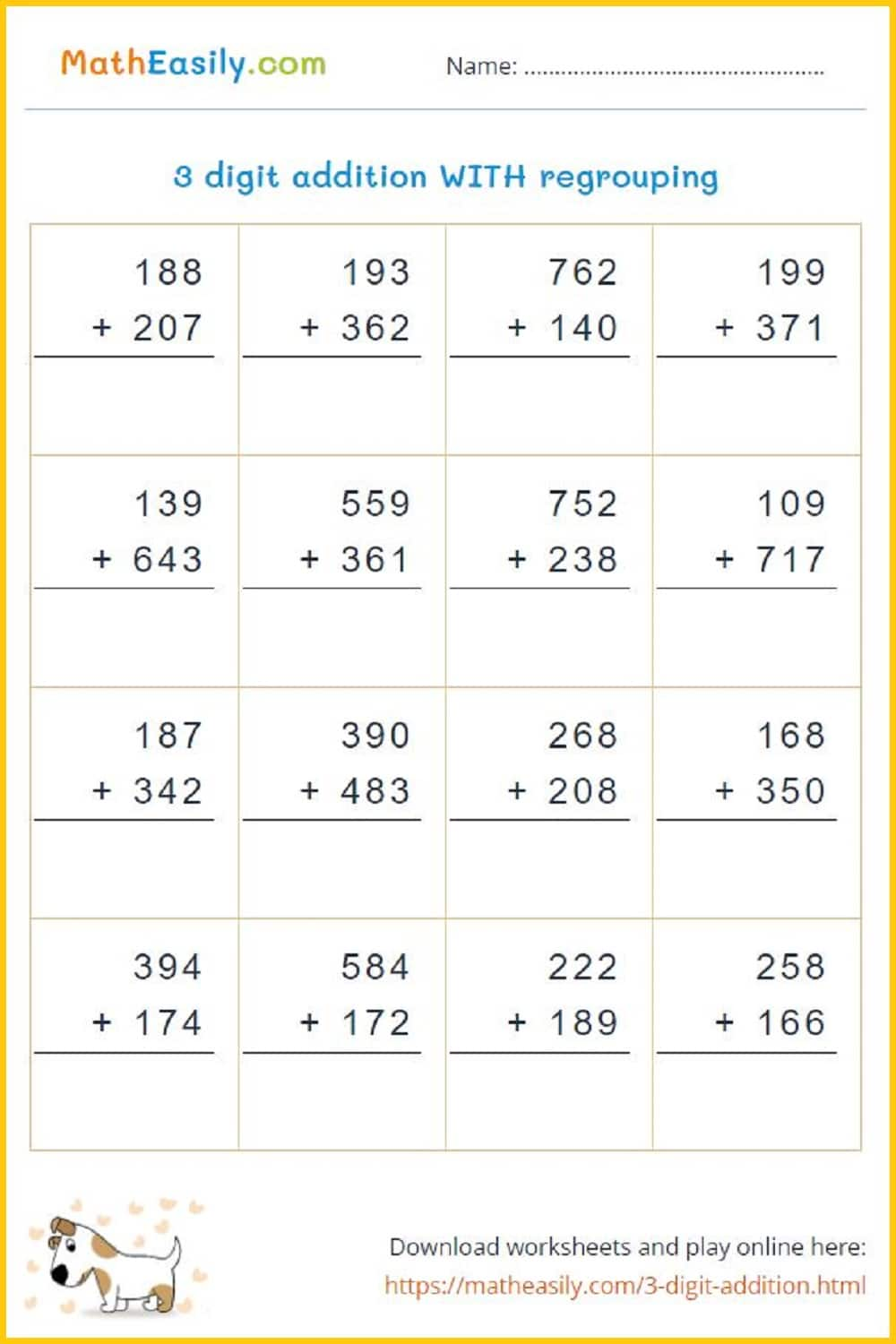Generate a new PDF

### 3 digit subtraction

3 digit subtraction WITHOUT regrouping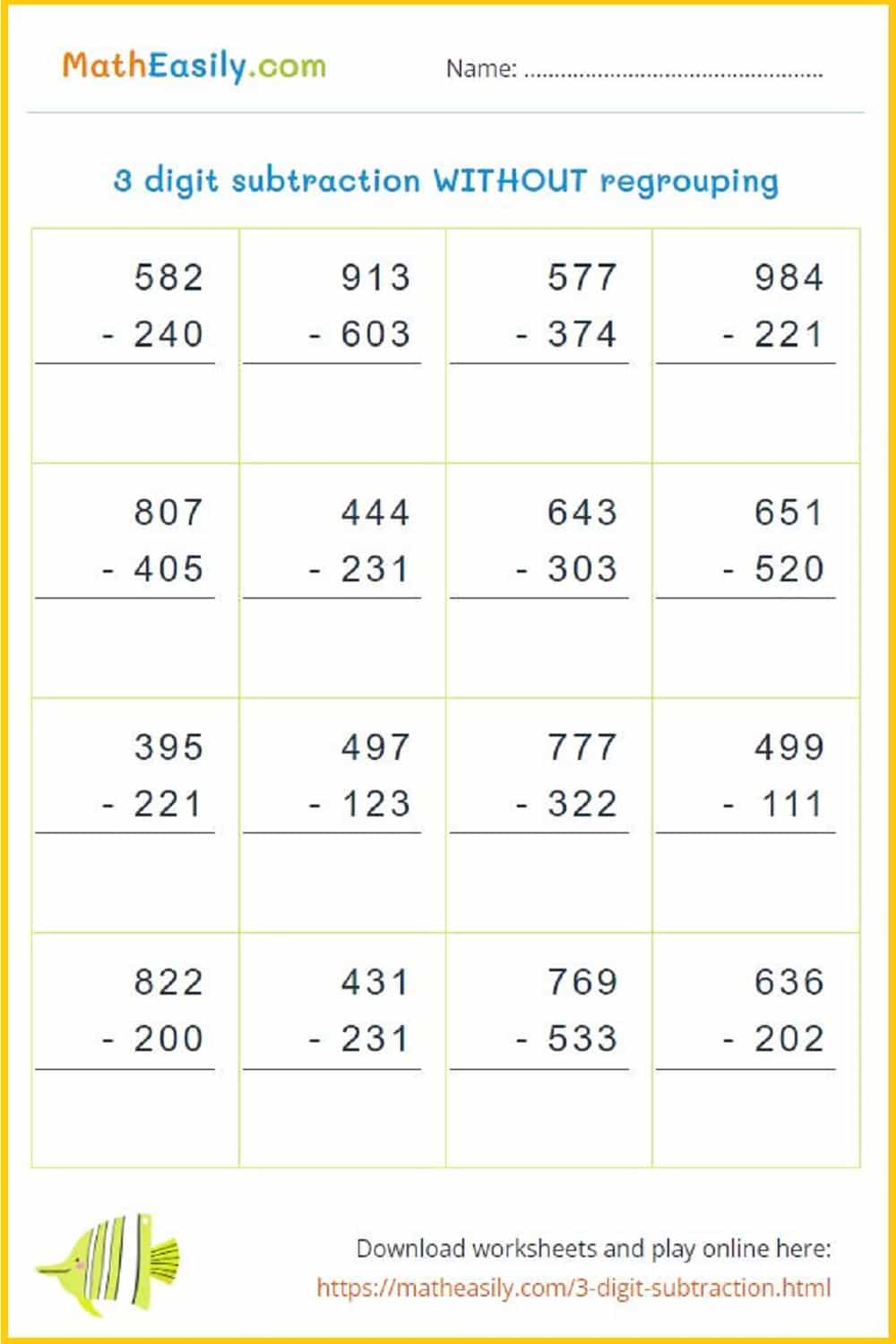Generate a new PDF

### 3 digit subtraction

3 digit subtraction worksheets WITH regroupingGenerate a new PDF

##### 100 Matchstick Puzzles in PDF

Printable math matchstick puzzles with answers. Move 1 stick to fix the equation.

US\$ 7.00

LOOK INSIDE

## Math Worksheets for 3rd Grade MULTIPLICATION

Our printable multiplication worksheets for Grade 3 help kids to master multiplication. These free printable 3rd grade worksheets are prepared with the aim of to help students learn math in a easy way.

### Multiplication board game

Learning multiplication is one of the most challenging tasks in math. To help our kids to learn it easily I designed this printable multiplication board game in PDF.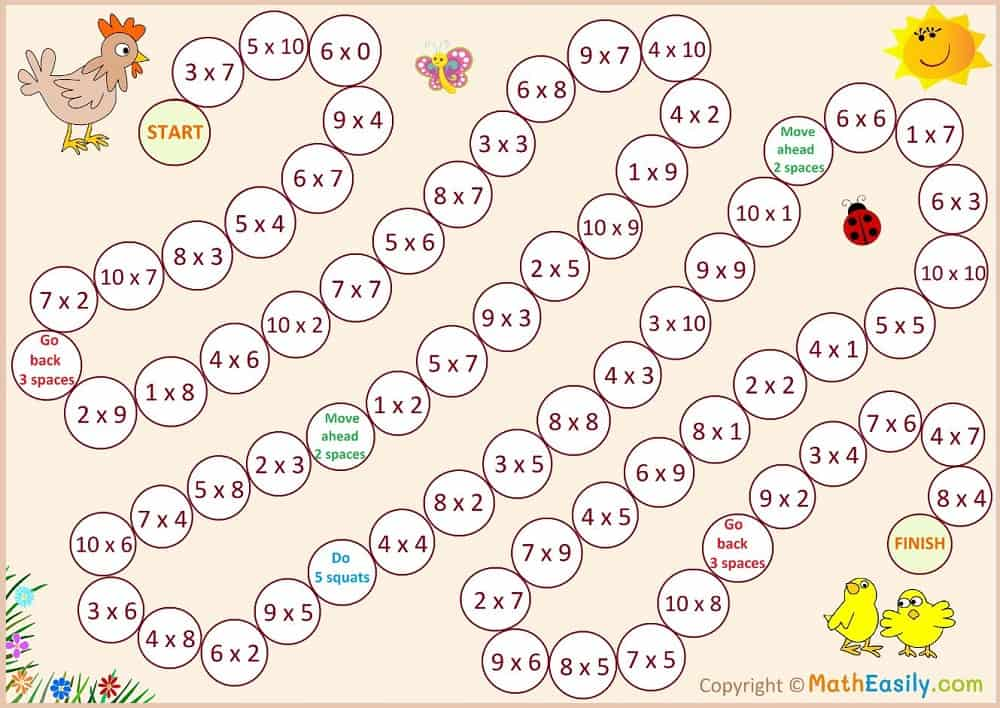### Multiplication flash cards

Practice multiplication 1-12 with these free printable multiplication flash cards in PDF.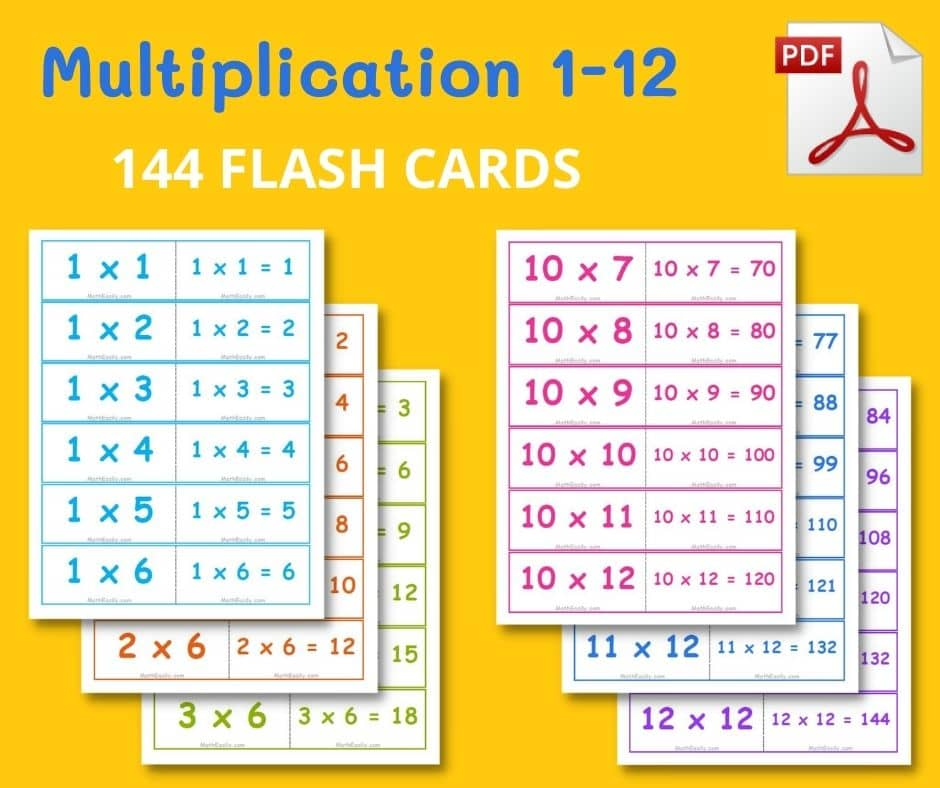### Multiplication puzzles

Puzzles are automatically generated, so they are different every time.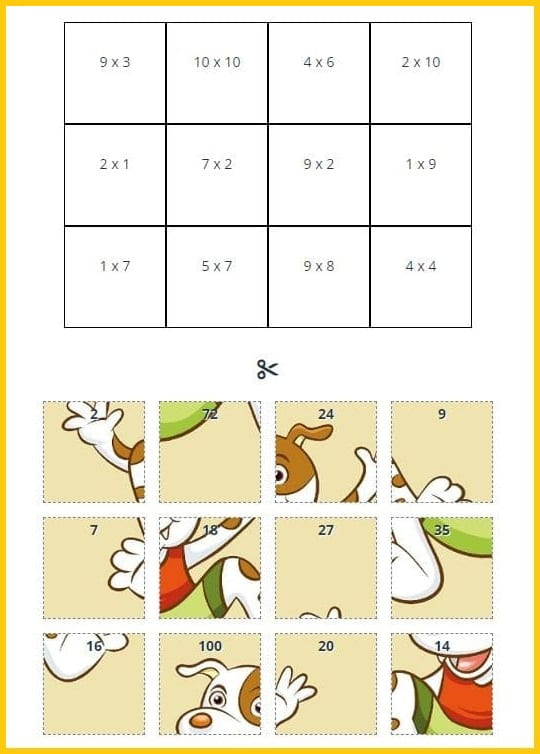### Single digit multiplication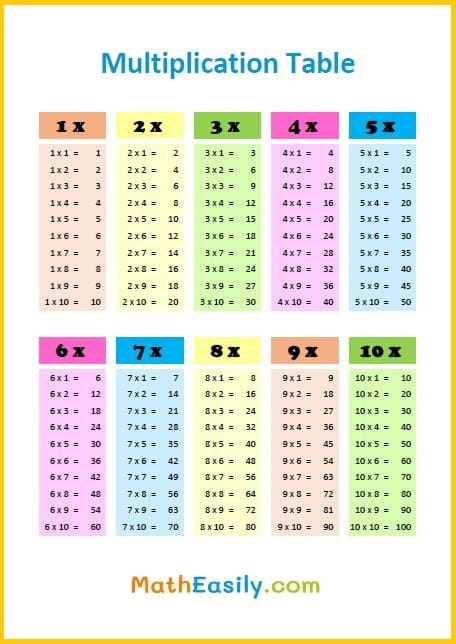### Multiplication dice game

Play easy multiplication game with dice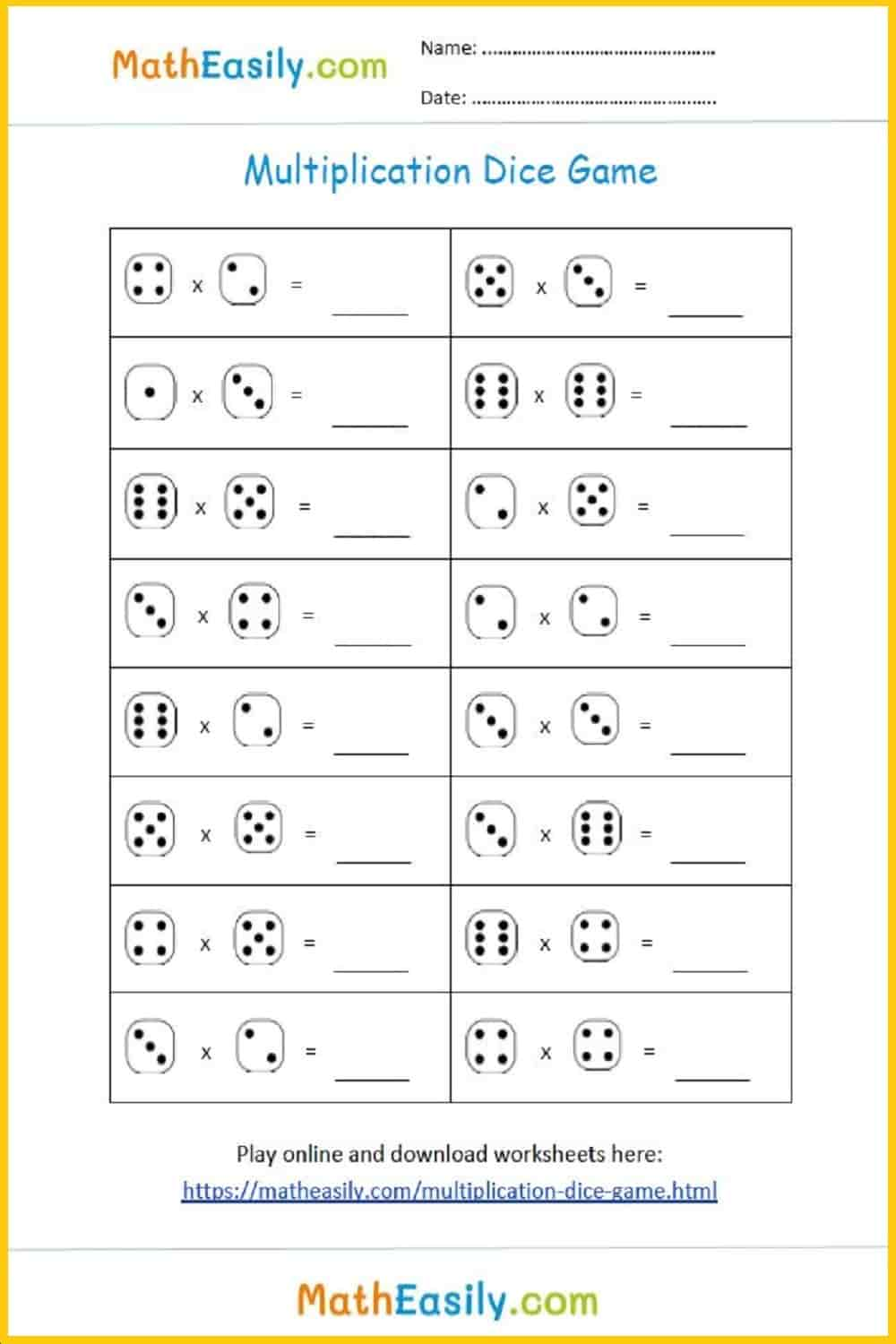### Single digit multiplication

Practice single digit multiplication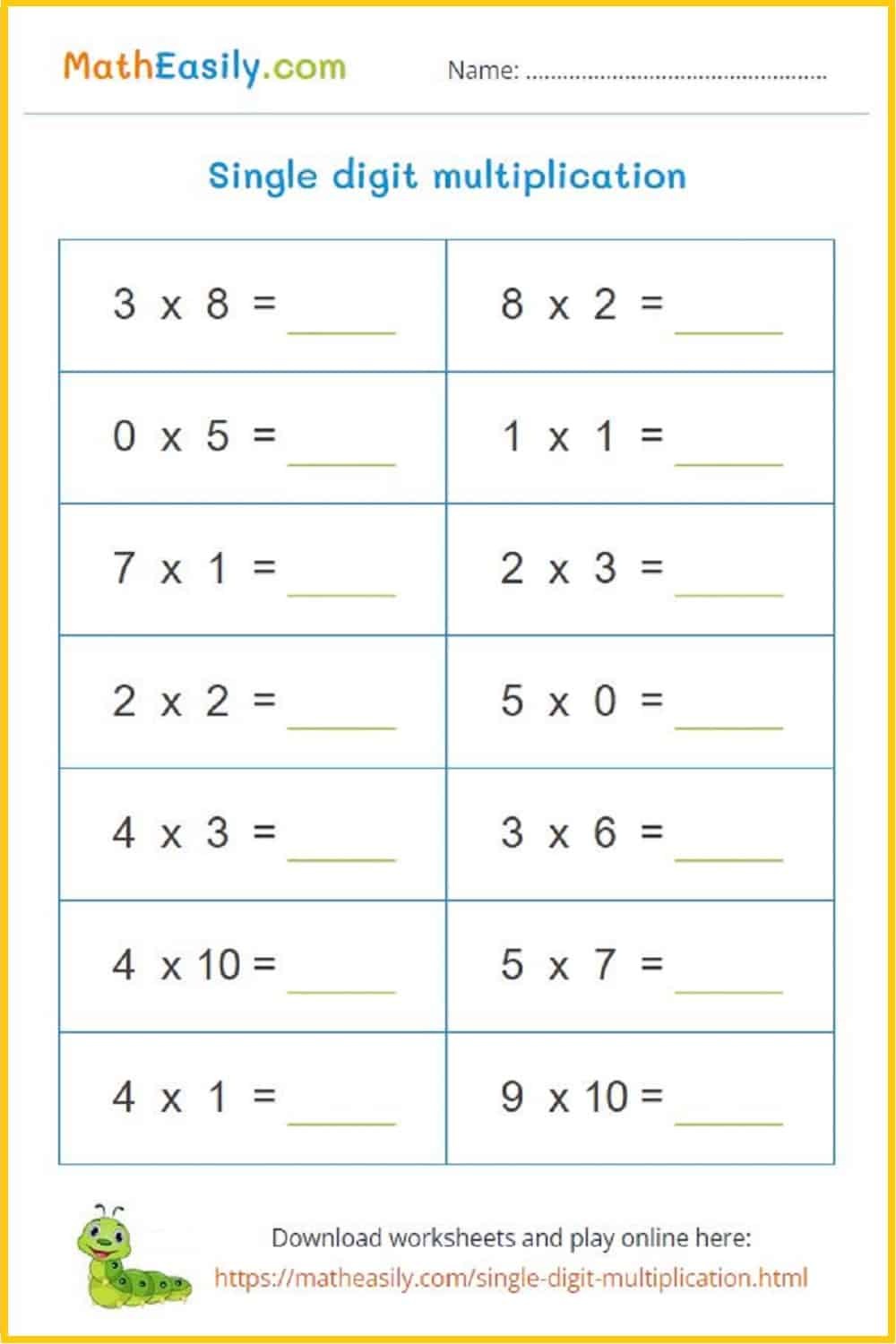Generate a new PDF

### Multiplication chart

Free printable multiplication chart 1-12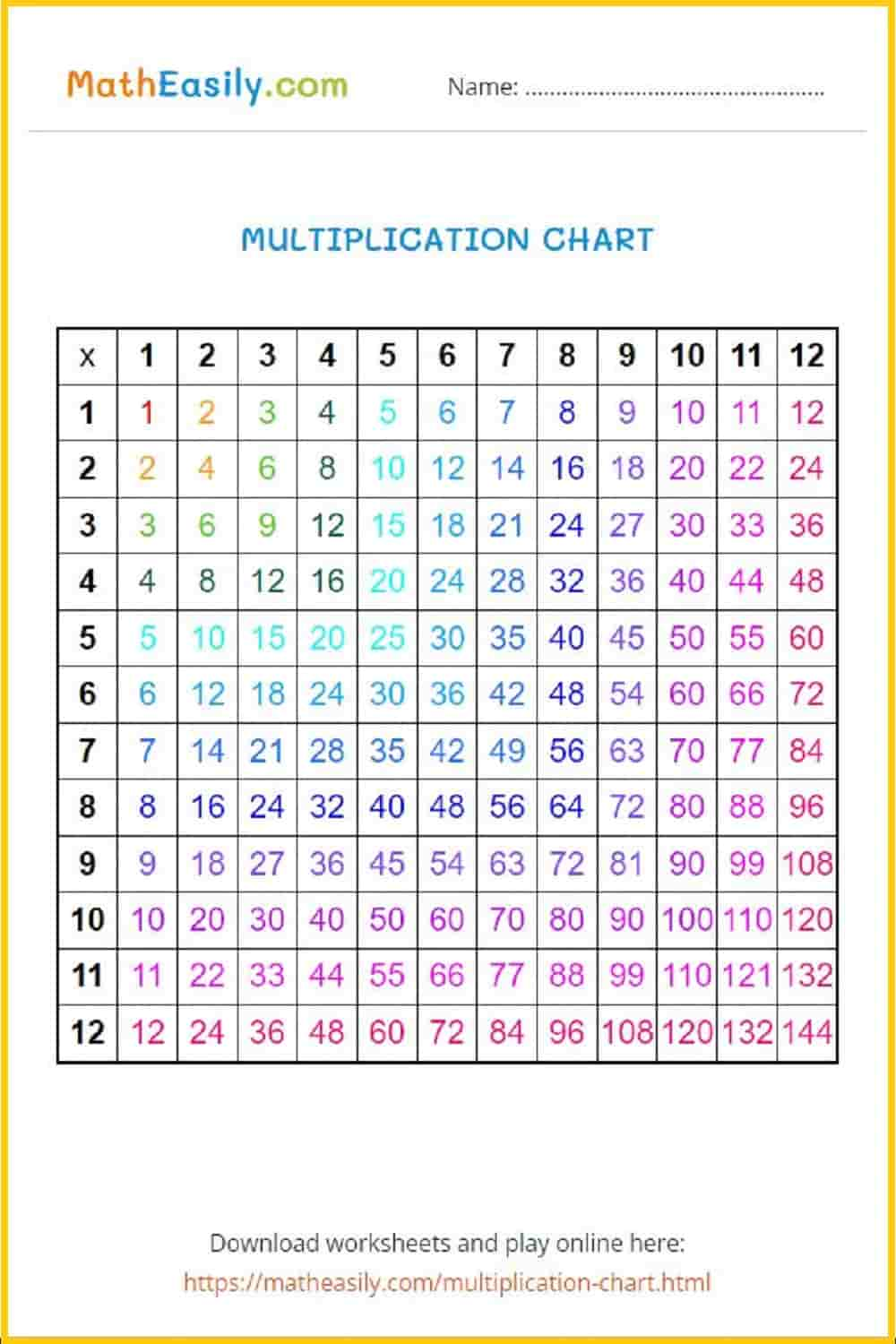### Multiplication chart

Multiplication chart with missing numbers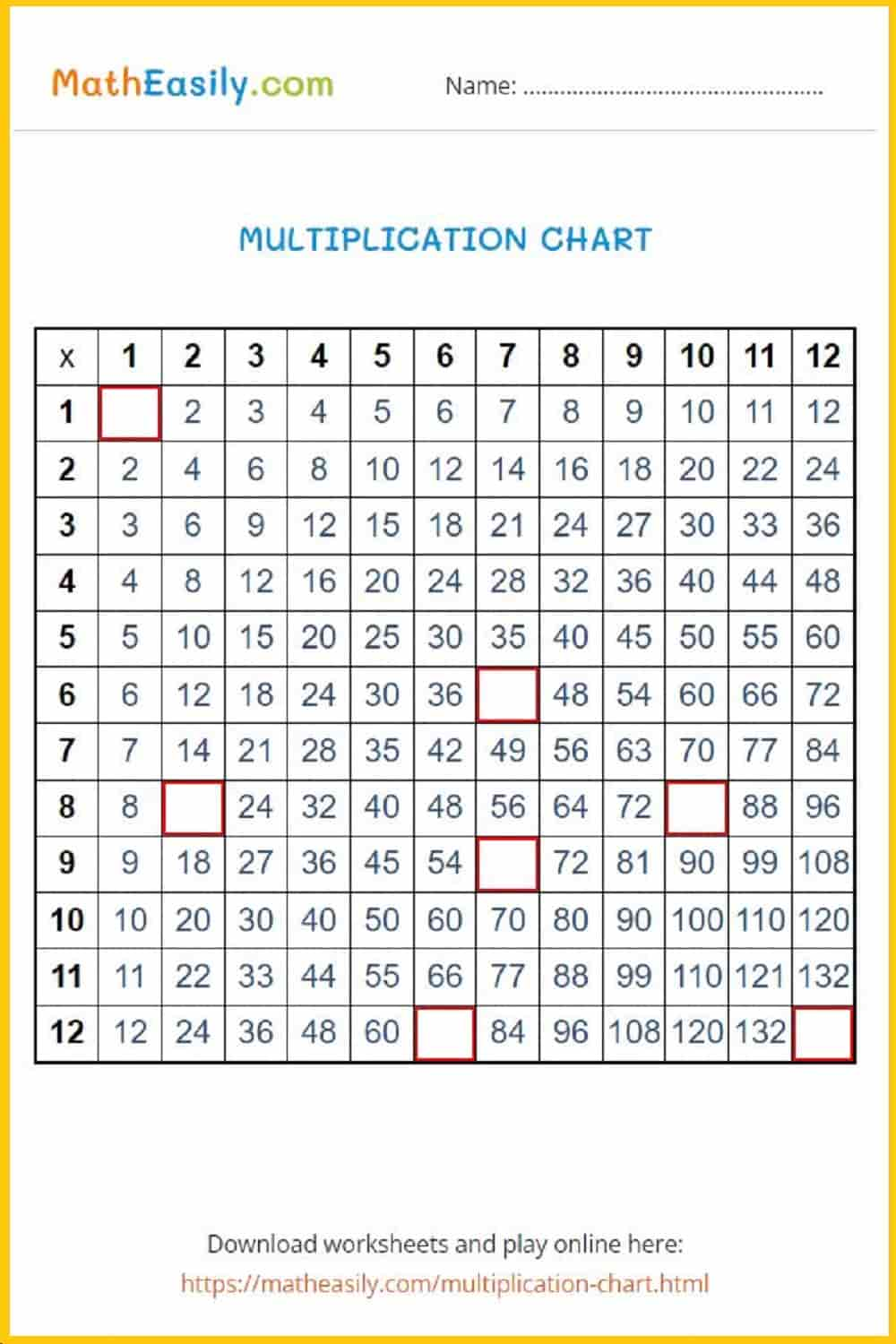Generate a new PDF

### Multiplication chart

Blank multiplication chart in PDF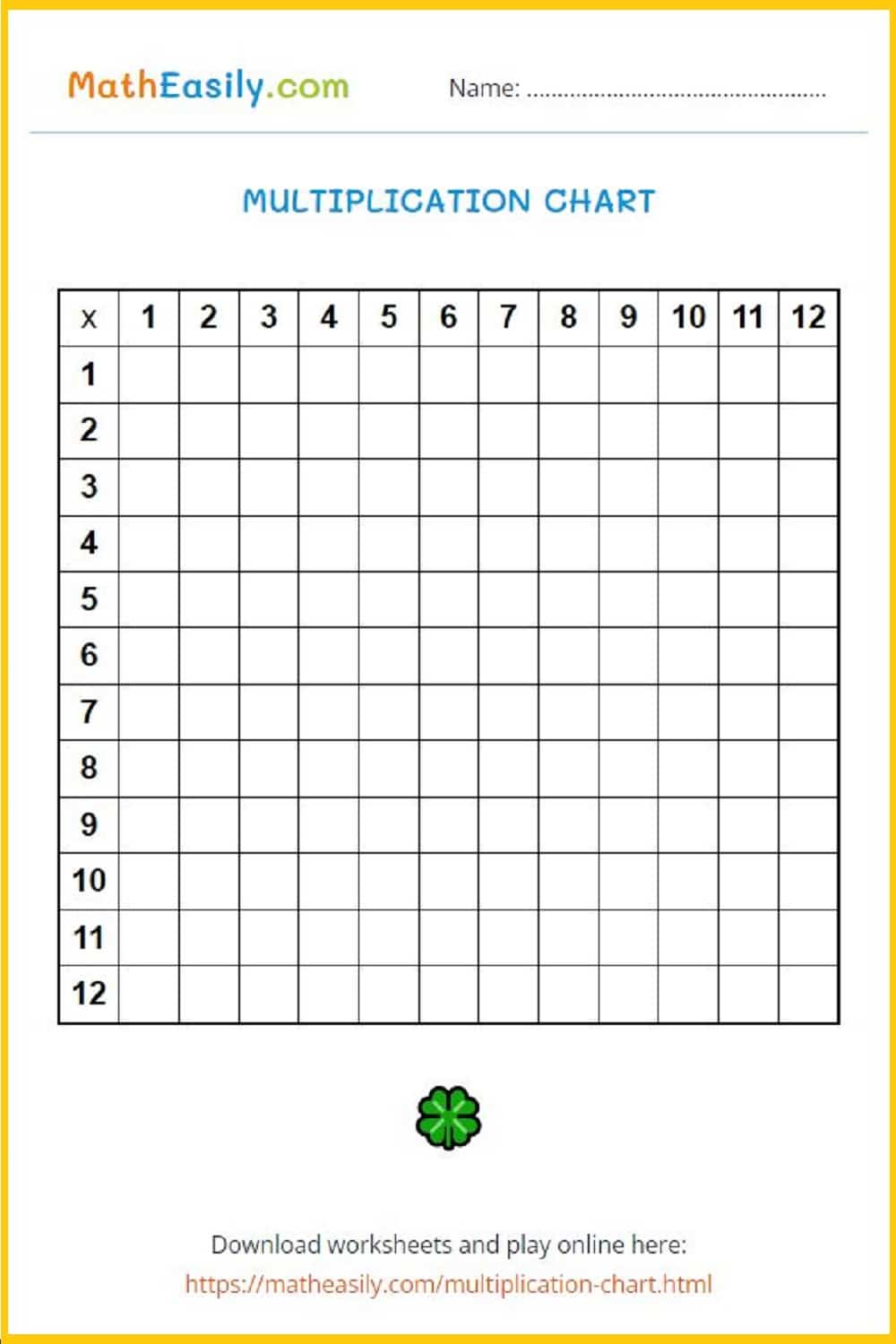### Multiplication pyramids

Multiplication pyramids to 100: worksheets with answersGenerate a new PDF

### Multiplication pyramids

Multiplication pyramids to 300: worksheets with answers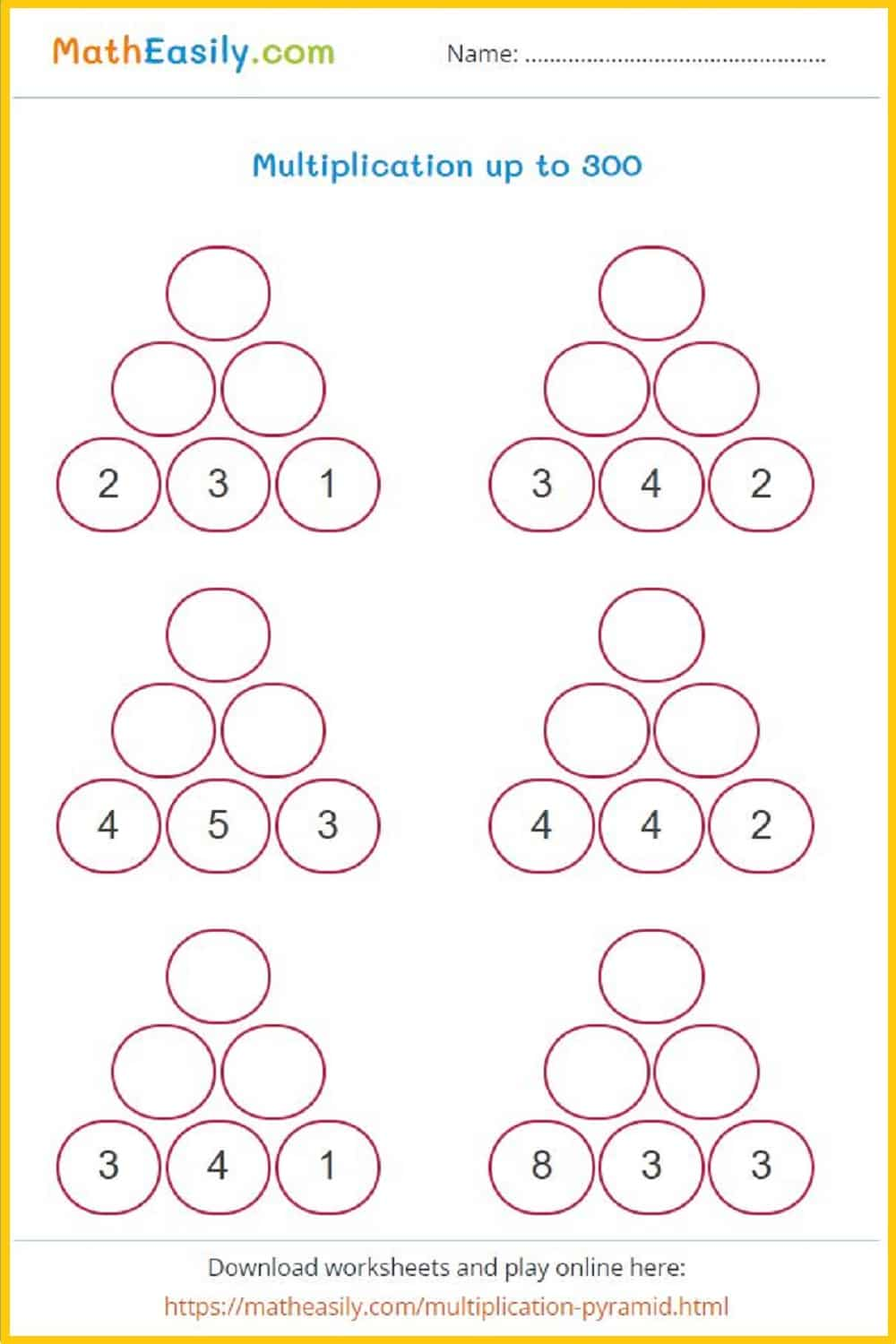Generate a new PDF

### Multiplication pyramids

Multiplication pyramids to 500: worksheets with answers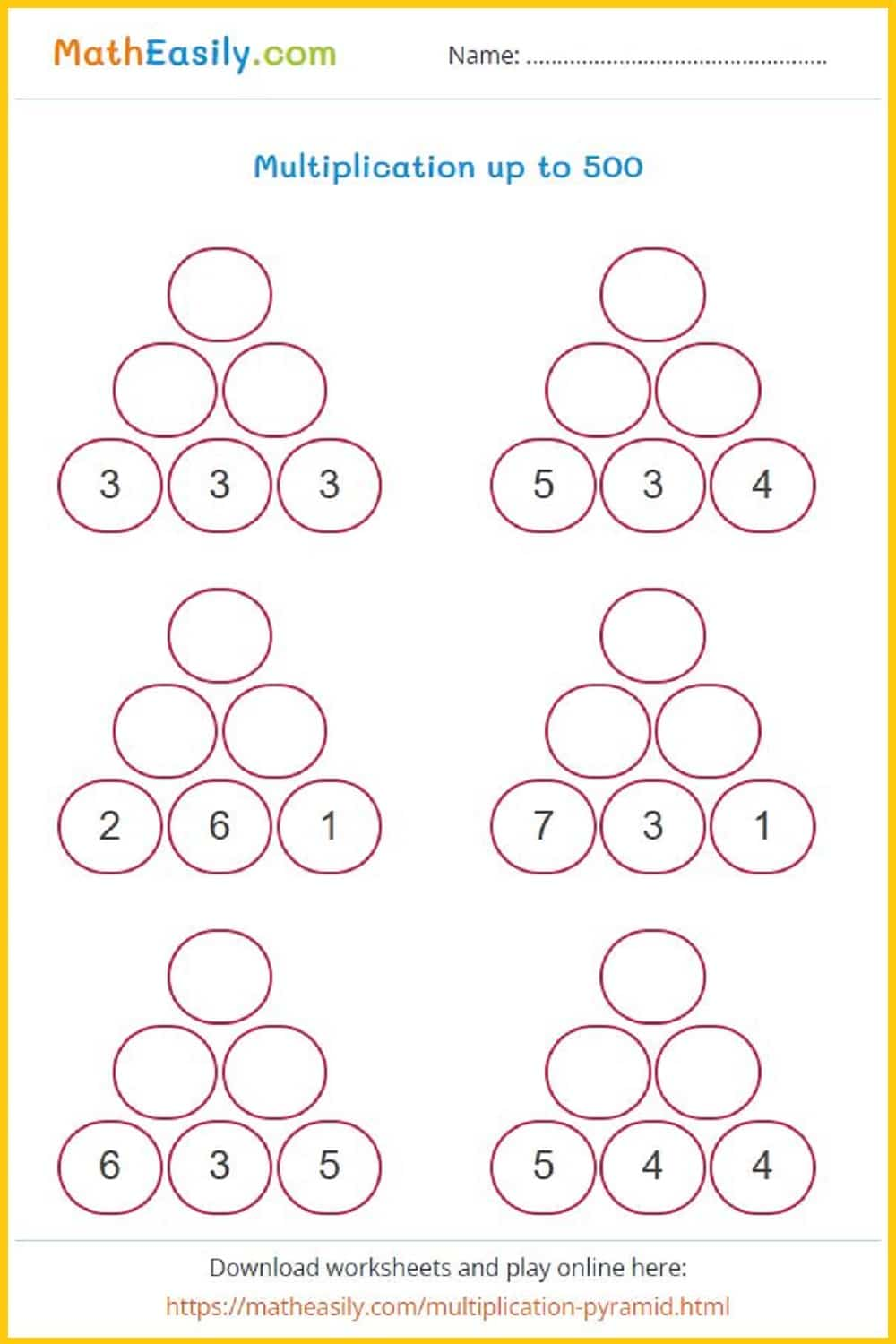Generate a new PDF

### Multiplication comparison

Multiply and compare the products: worksheets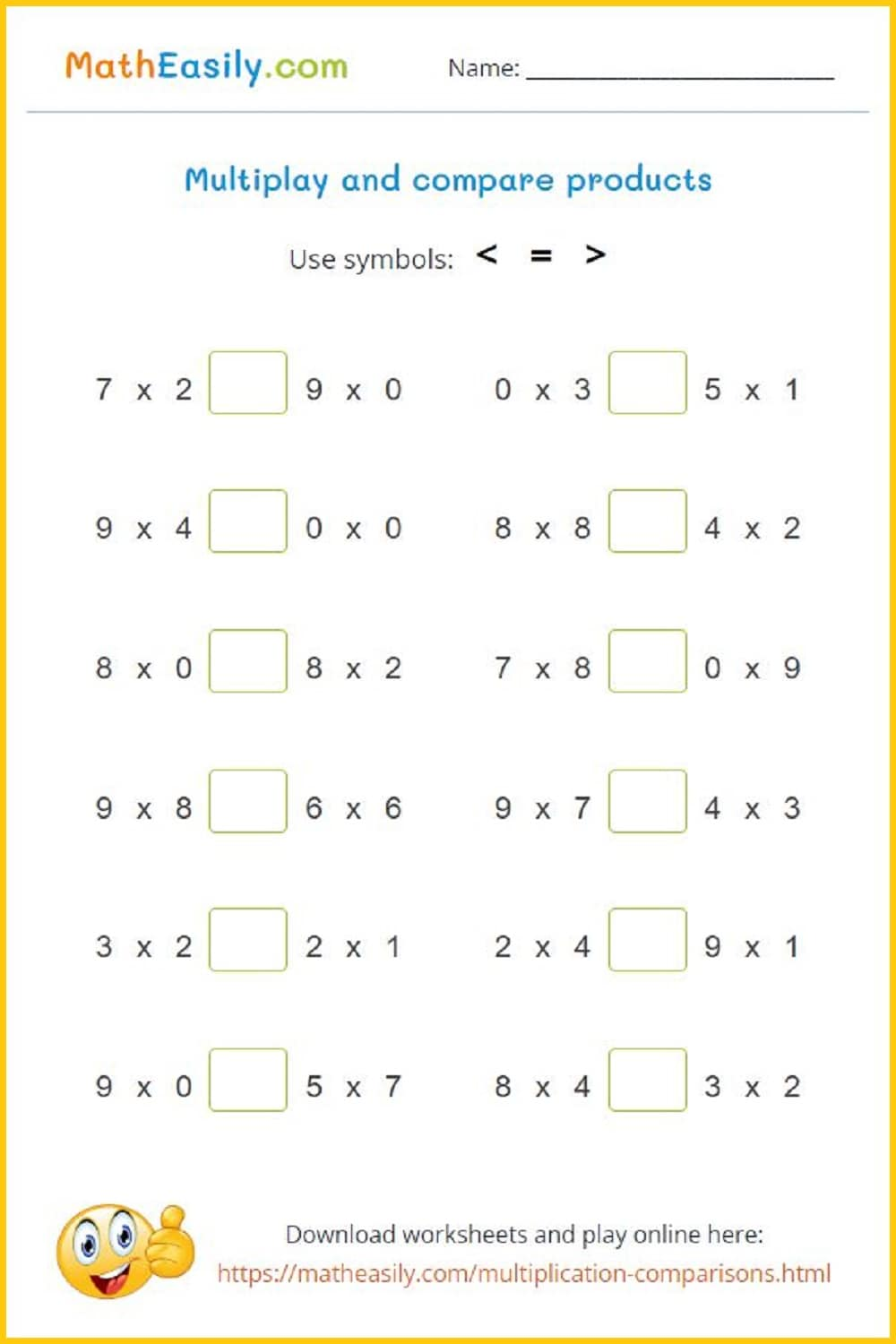Generate a new PDF

### Multiply 2 digit by 1 digit

2 digit by 1 digit multiplication worksheets with answers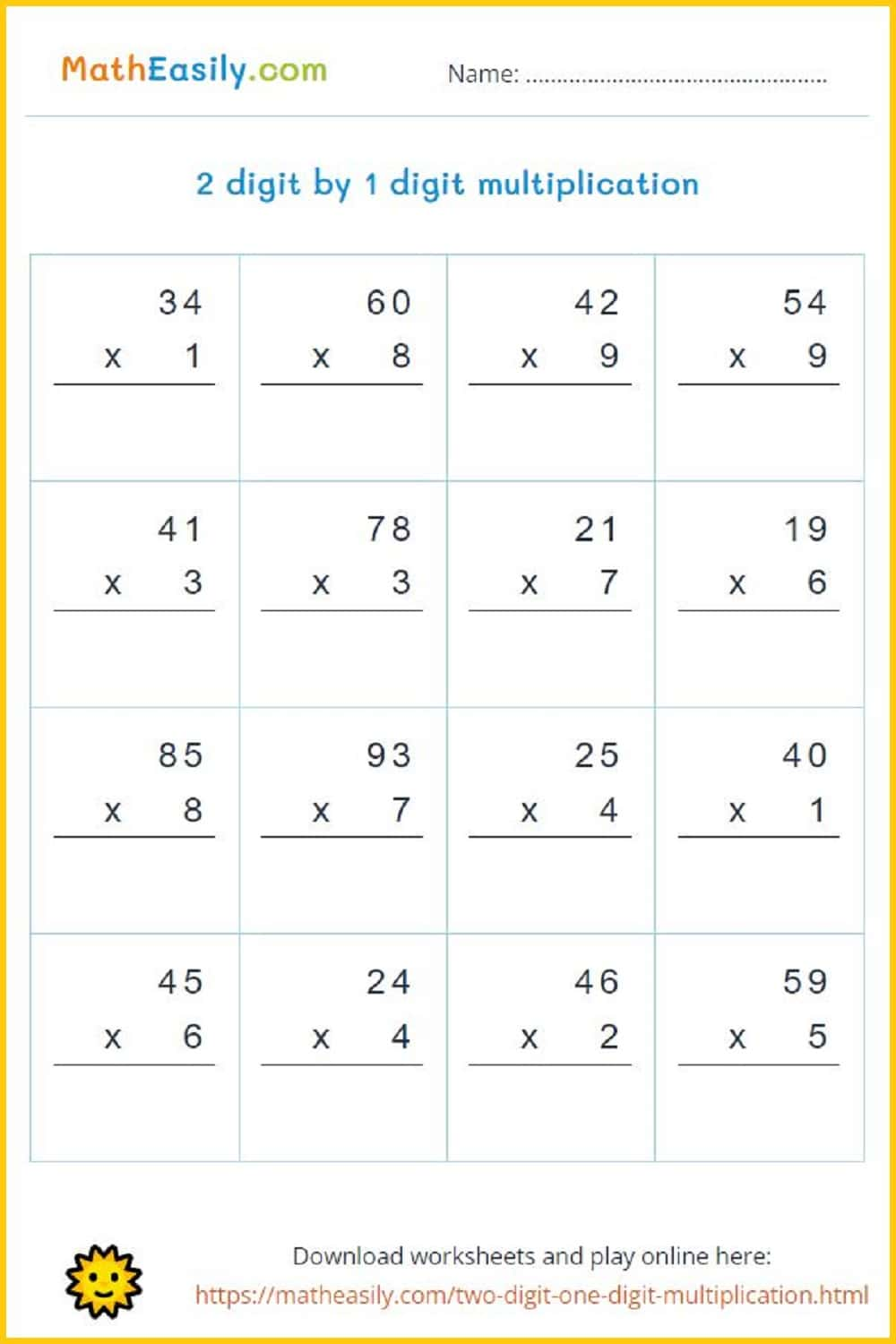Generate a new PDF

### Multiply 3 digit by 1 digit

3 digit by 1 digit multiplication worksheets with answers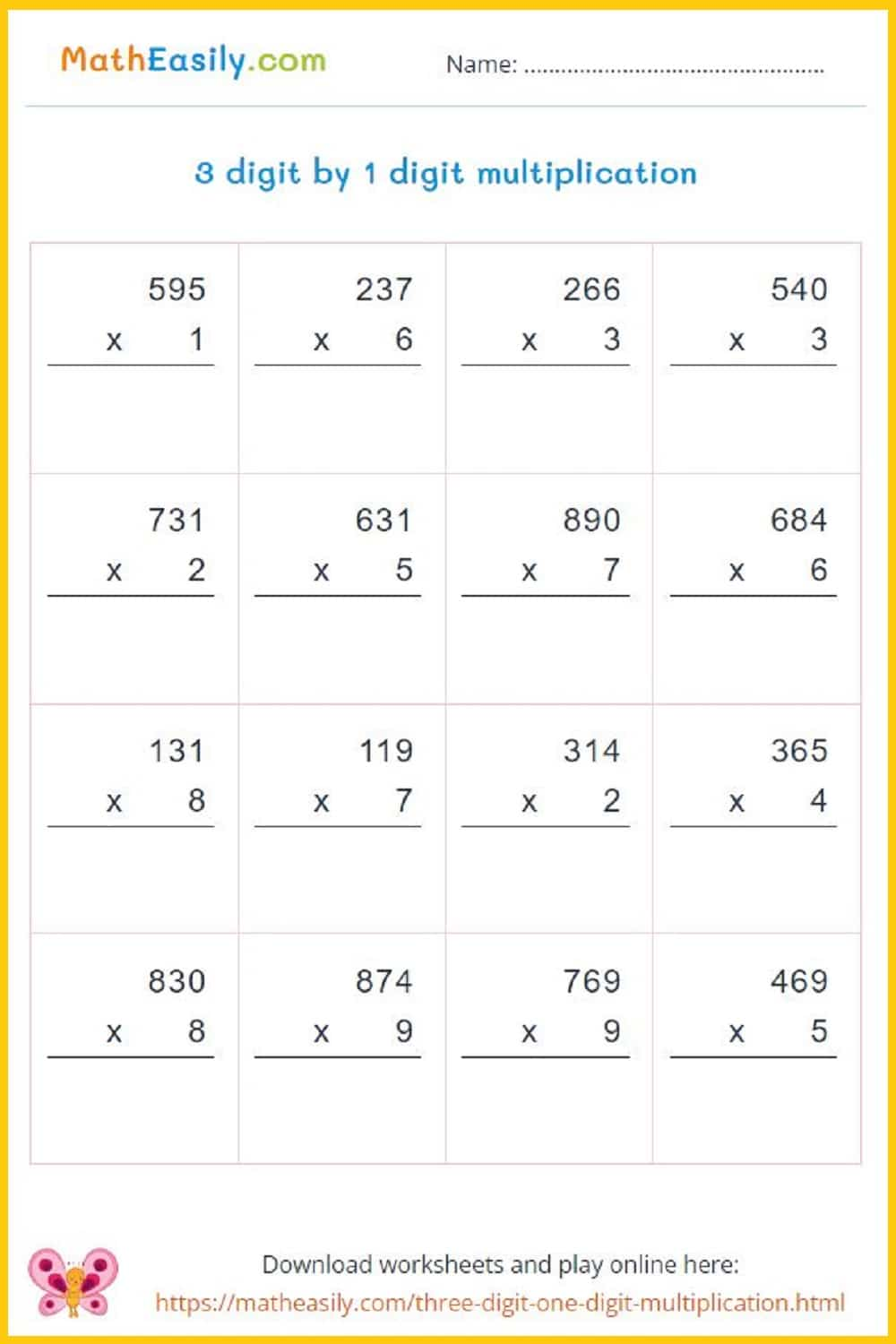Generate a new PDF

### Multiply 4 digit by 1 digit

4 digit by 1 digit multiplication worksheets with answers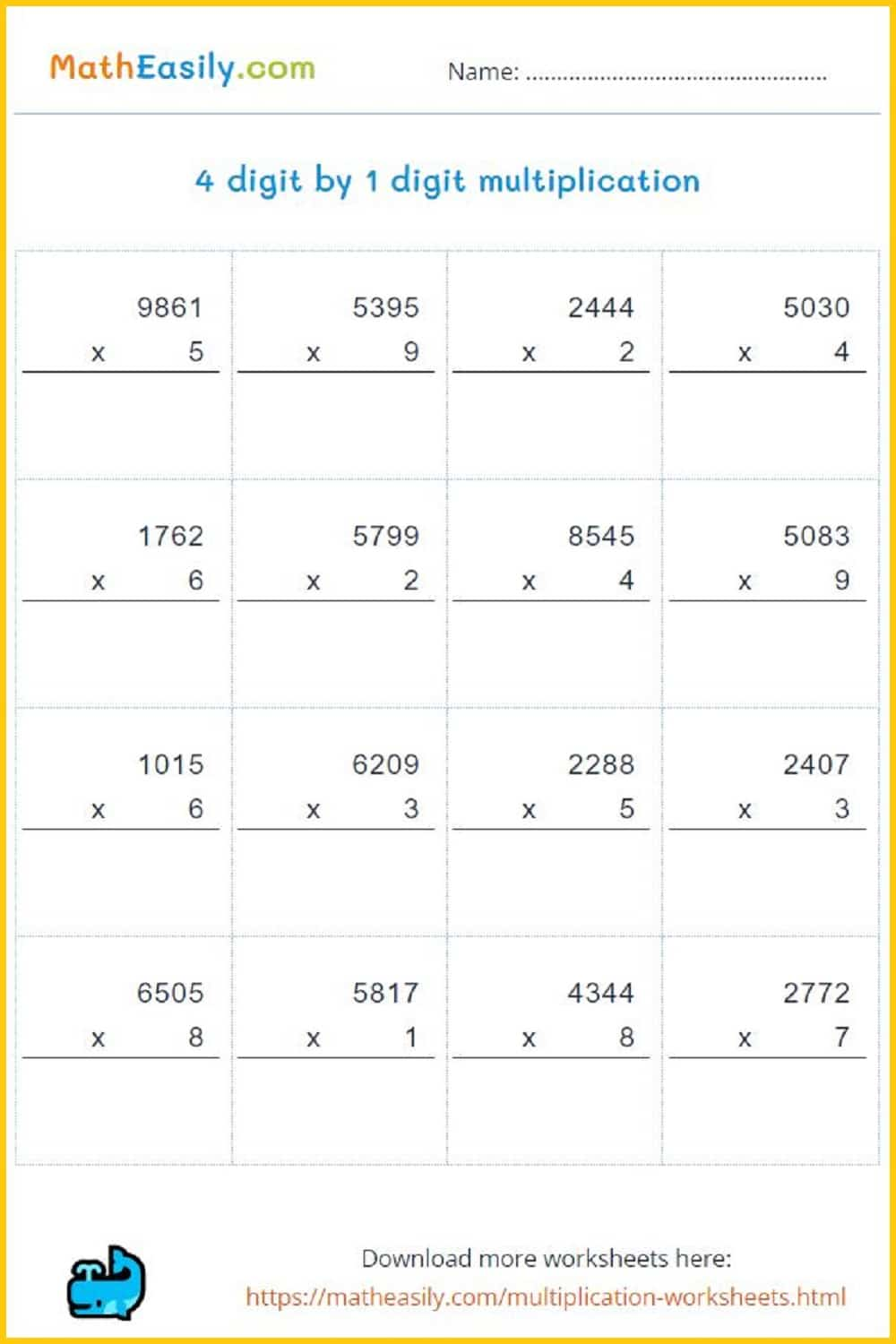Generate a new PDF

### 2 digit by 2 digit

Multiply 2 digit by 2 digit numbers: worksheets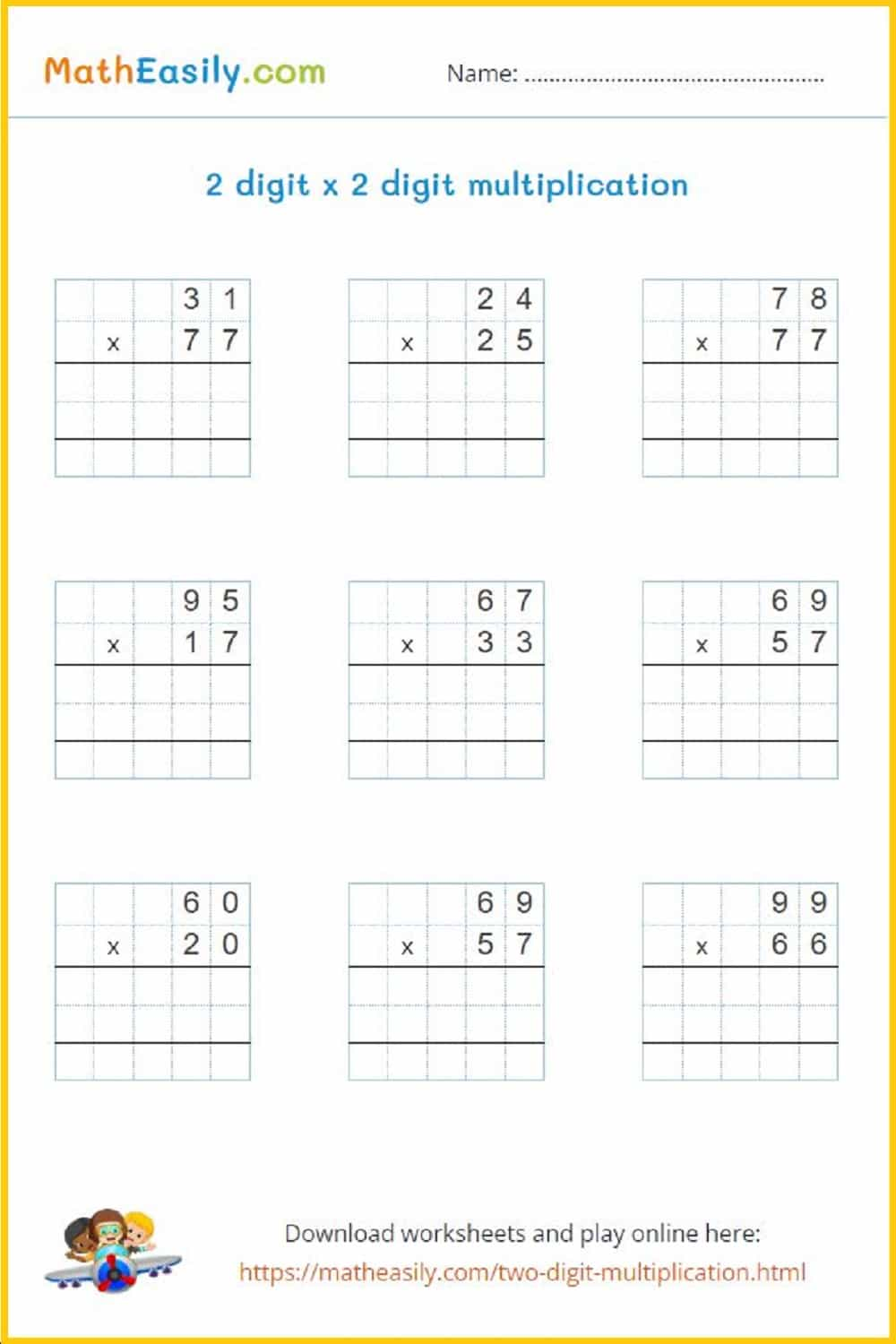Generate a new PDF

#### Multiplication games in PDF

50 multiplication puzzles

Fun printable puzzles to practice multiplication. 50 different pictures, many multiplication tasks.

US\$ 3.00

## 3rd grade math worksheets DIVISION

Our free printable 3rd grade math division worksheets help kids to enjoy and master division. These math worksheets for 3rd grade are prepared with the aim of to help teachers and parents to teach division in a fun way.

### Division board game

Learning division is one of the most challenging tasks in math. Enjoy this printable division game in PDF.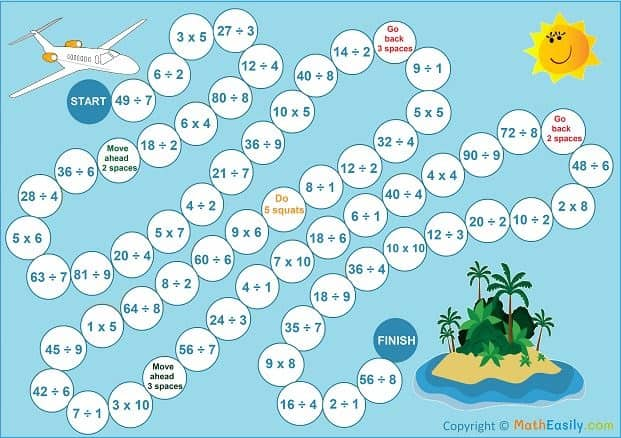### Division puzzles

Generate division worksheets, more than 50 pictures available.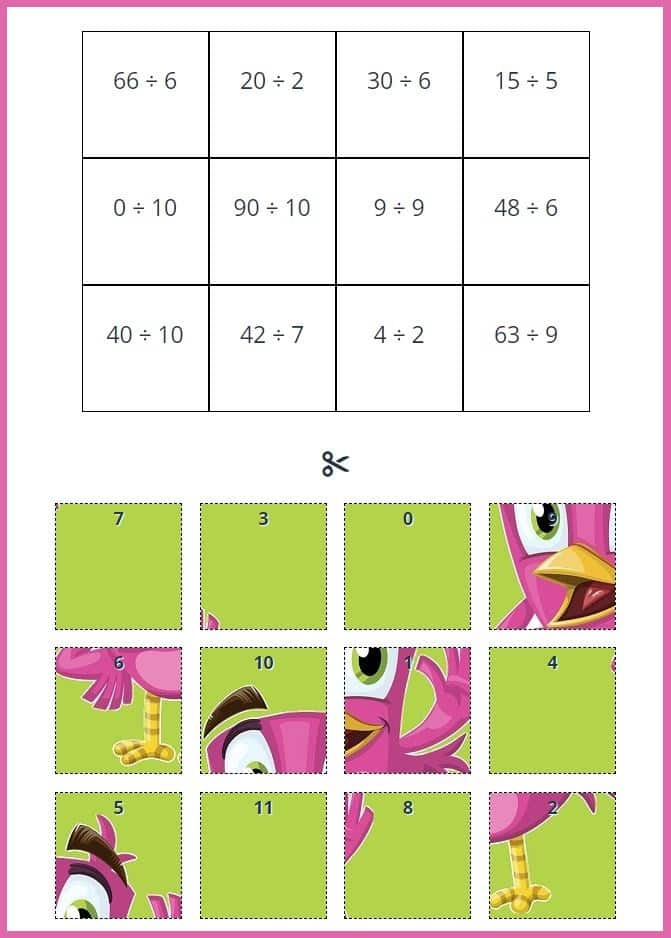Generate a new PDF

### Division

Single digit division worksheets with answers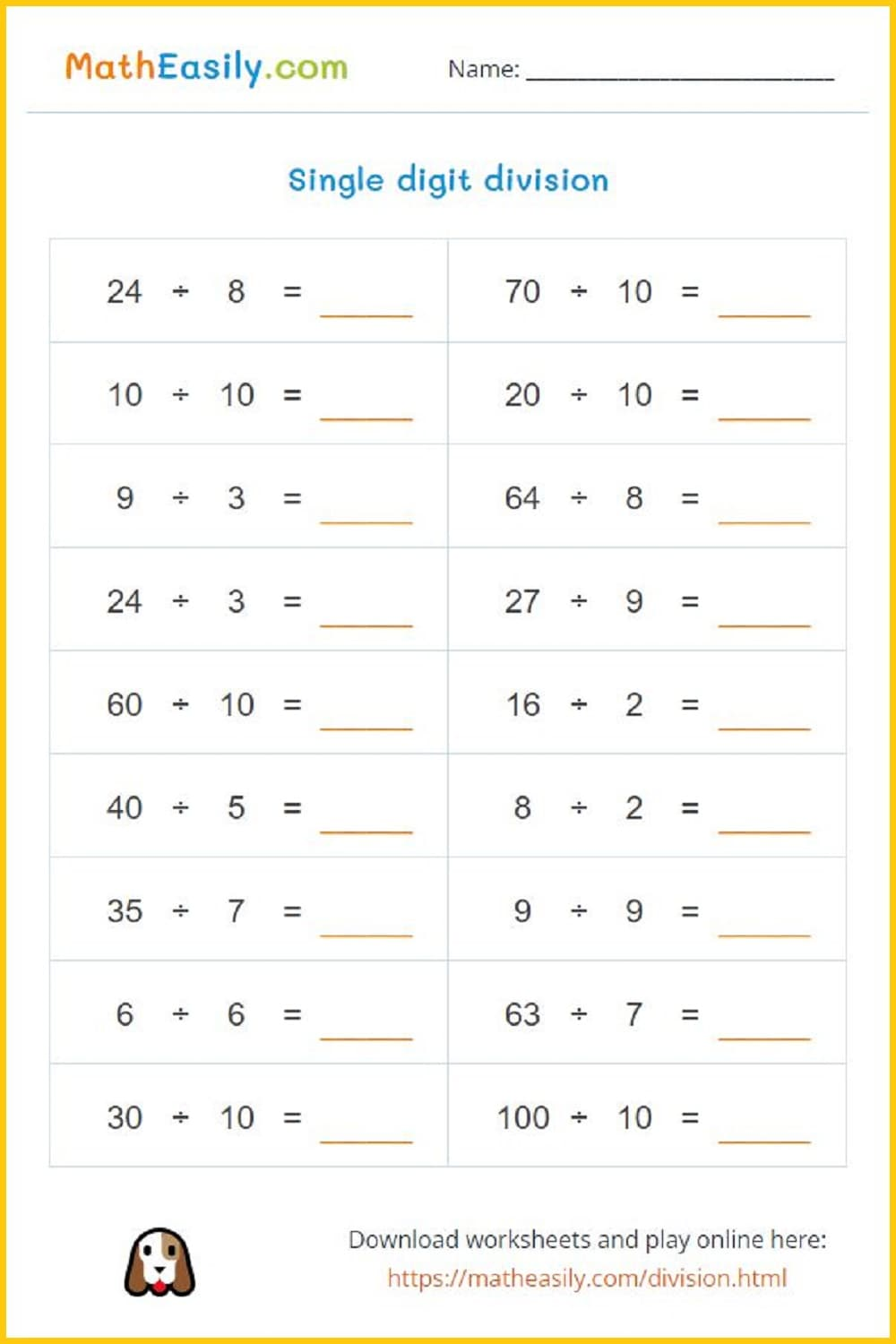Generate a new PDF

These grade 3 math worksheets cover addition, subtraction, multiplication, division and much more. You may freely use any of the printable math 3rd Grade Worksheets in the classroom or for homework. Just click on the math worksheet image and download the PDF. Our math worksheets for 3th grade are PDF documents with the answer key on the 2nd page.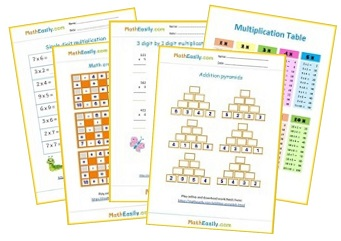Free Math Worksheets